# RS Aggarwal Solutions for Class 10 Maths Chapter 2 Polynomials

Get RS Aggarwal Solutions for Class 10 Chapter 2 Polynomials here. The subject experts at BYJU’S have solved each question of RS Aggarwal meticulously to help the students in solving any question from the book. Practice Polynomials RS Aggarwal questions and become master on the concepts. All solutions are explained using step-by-step approach. DownloadRS Aggarwal Maths Class 10 Solutions and score more in your exams.

## Download PDF of RS Aggarwal Solutions for Class 10 Chapter 2 Polynomials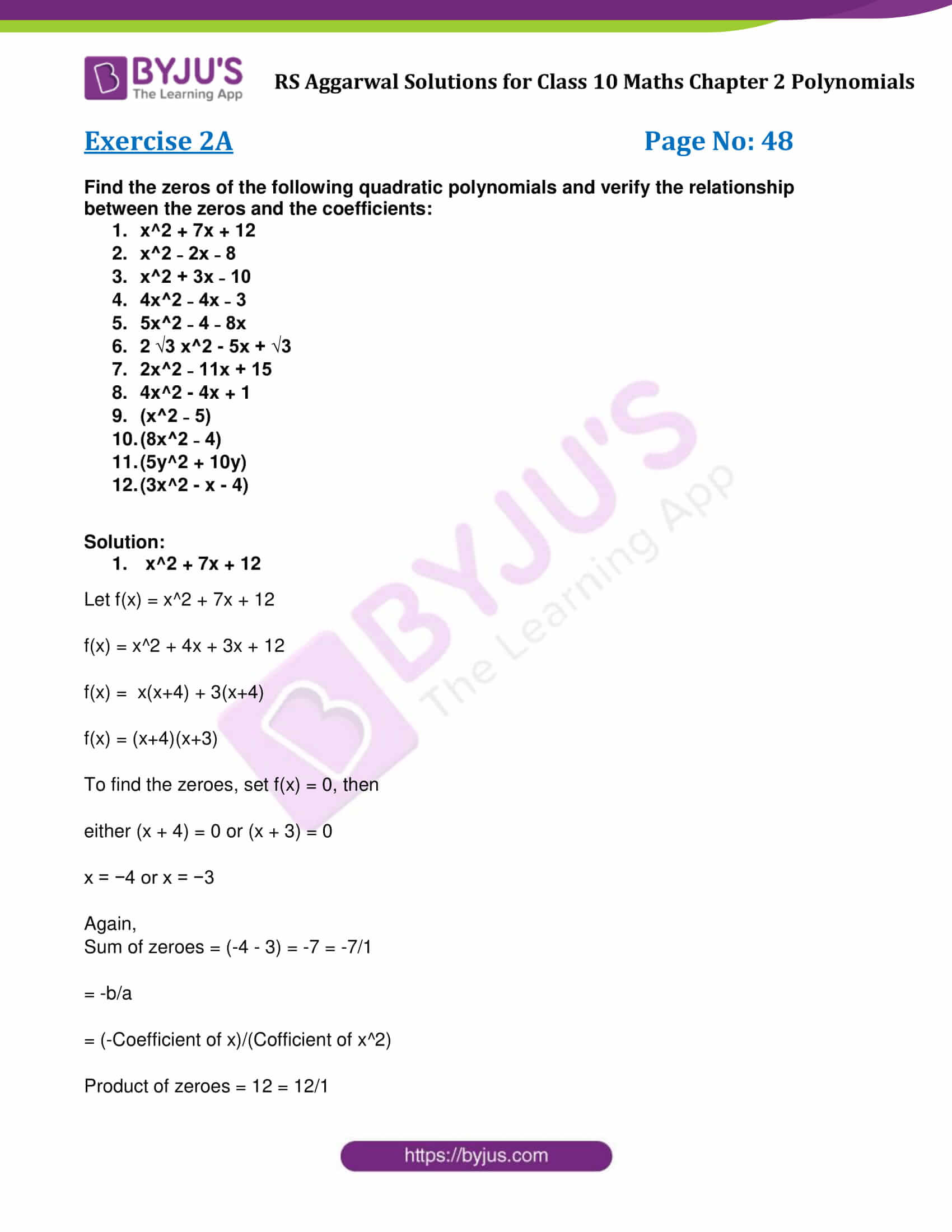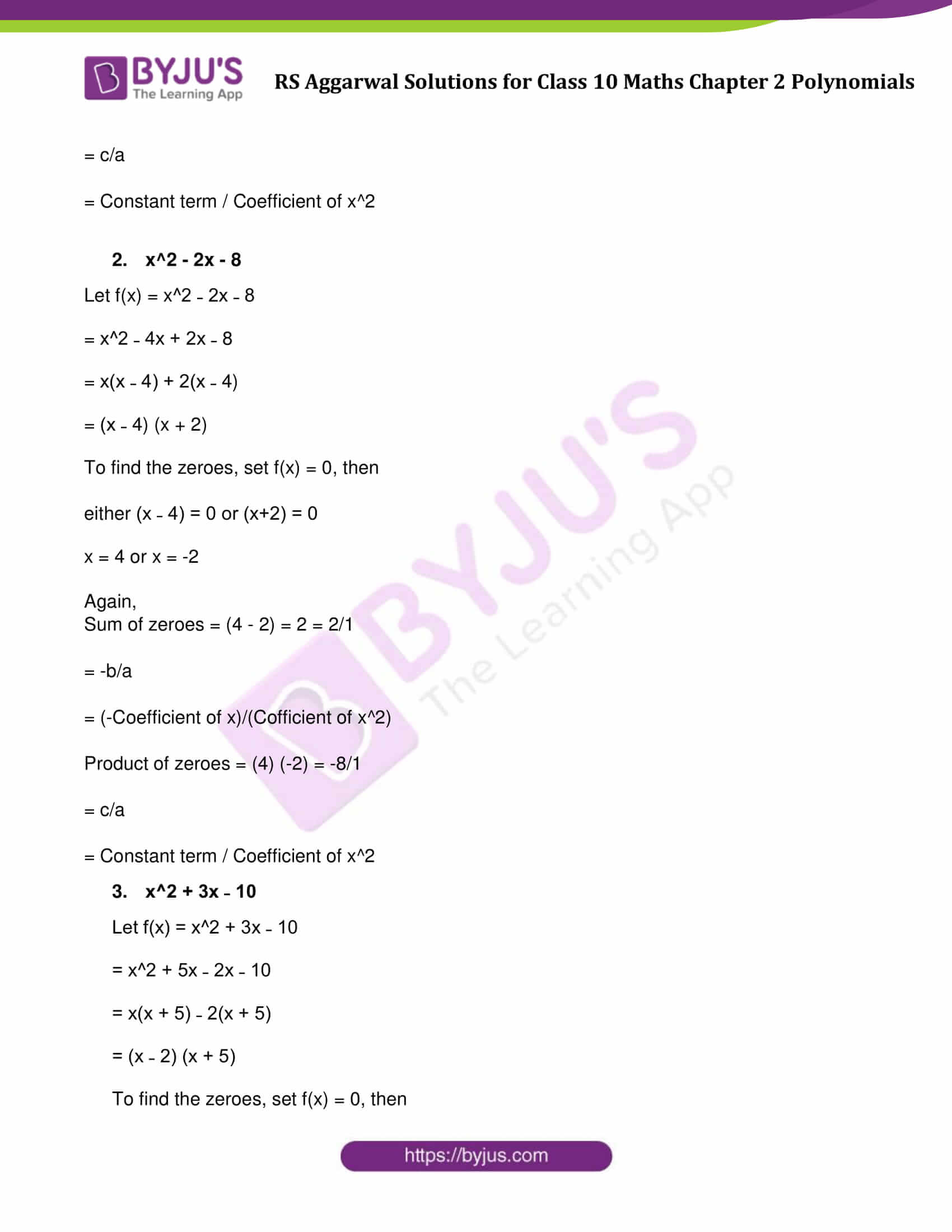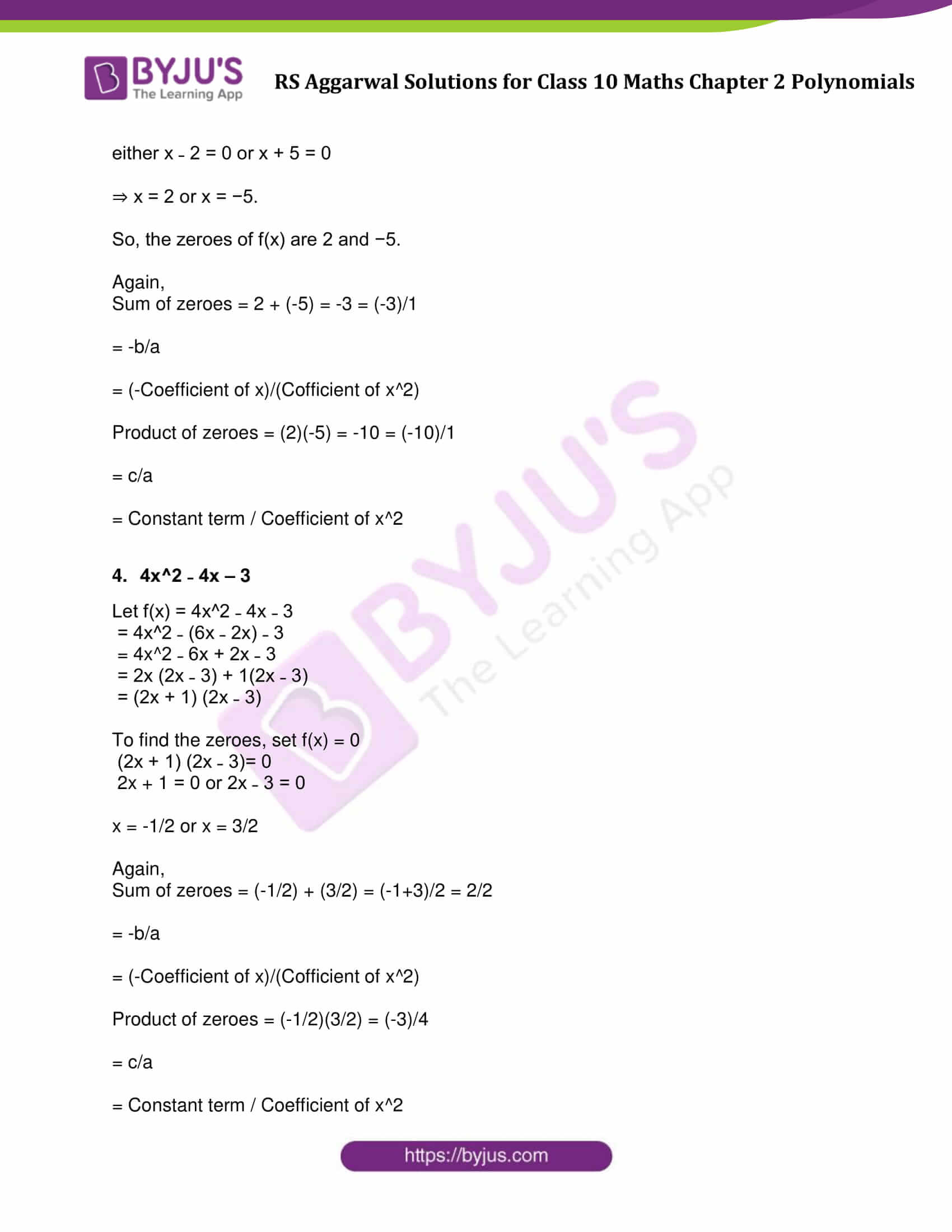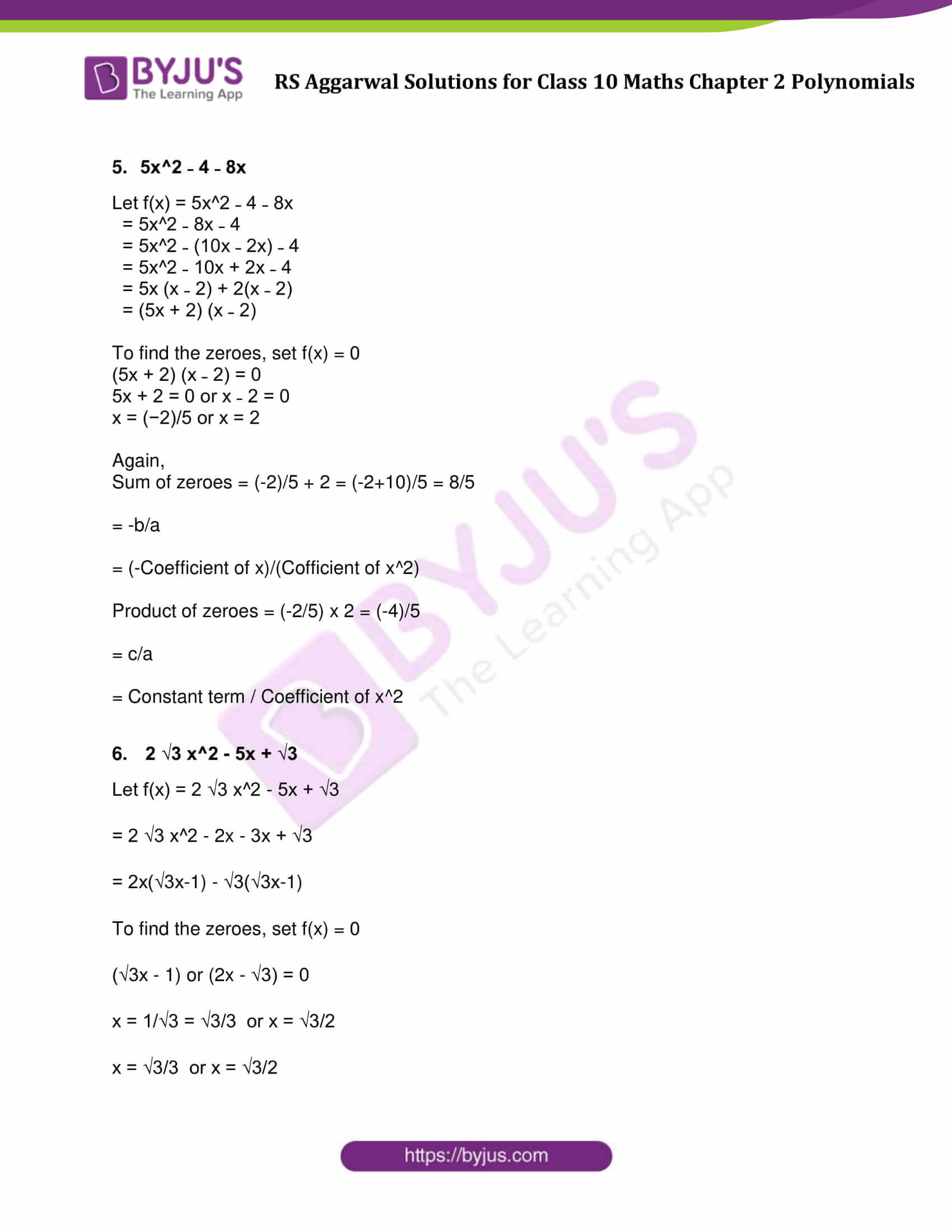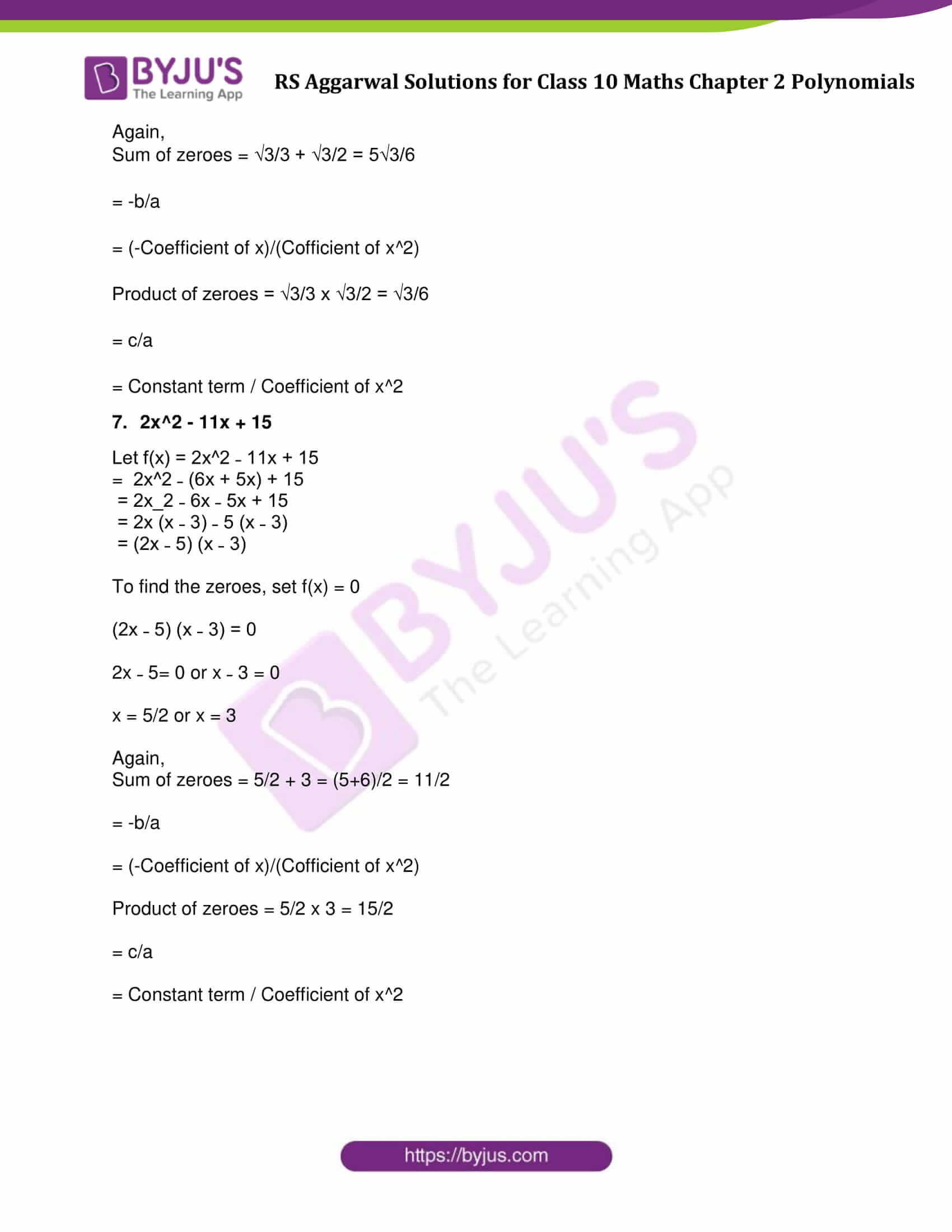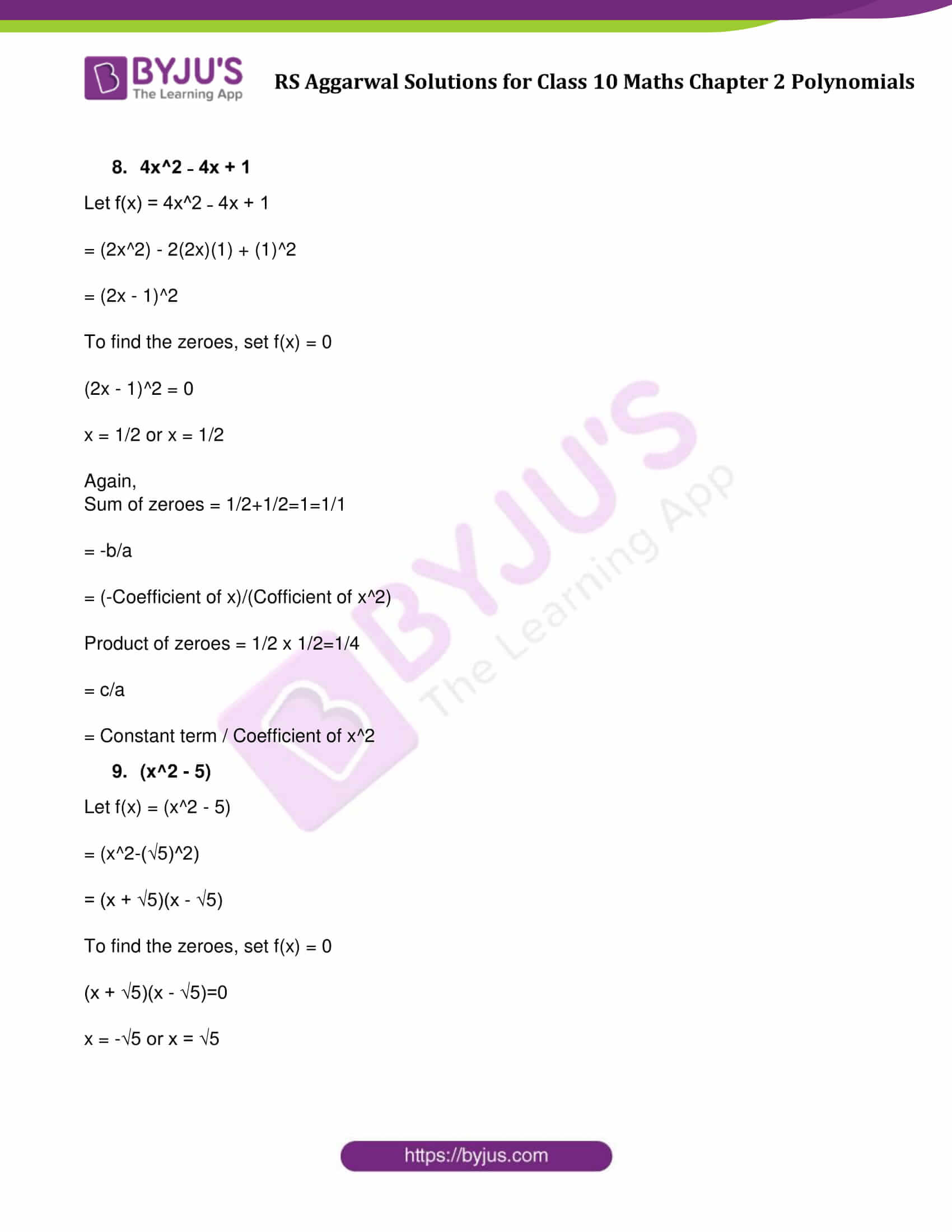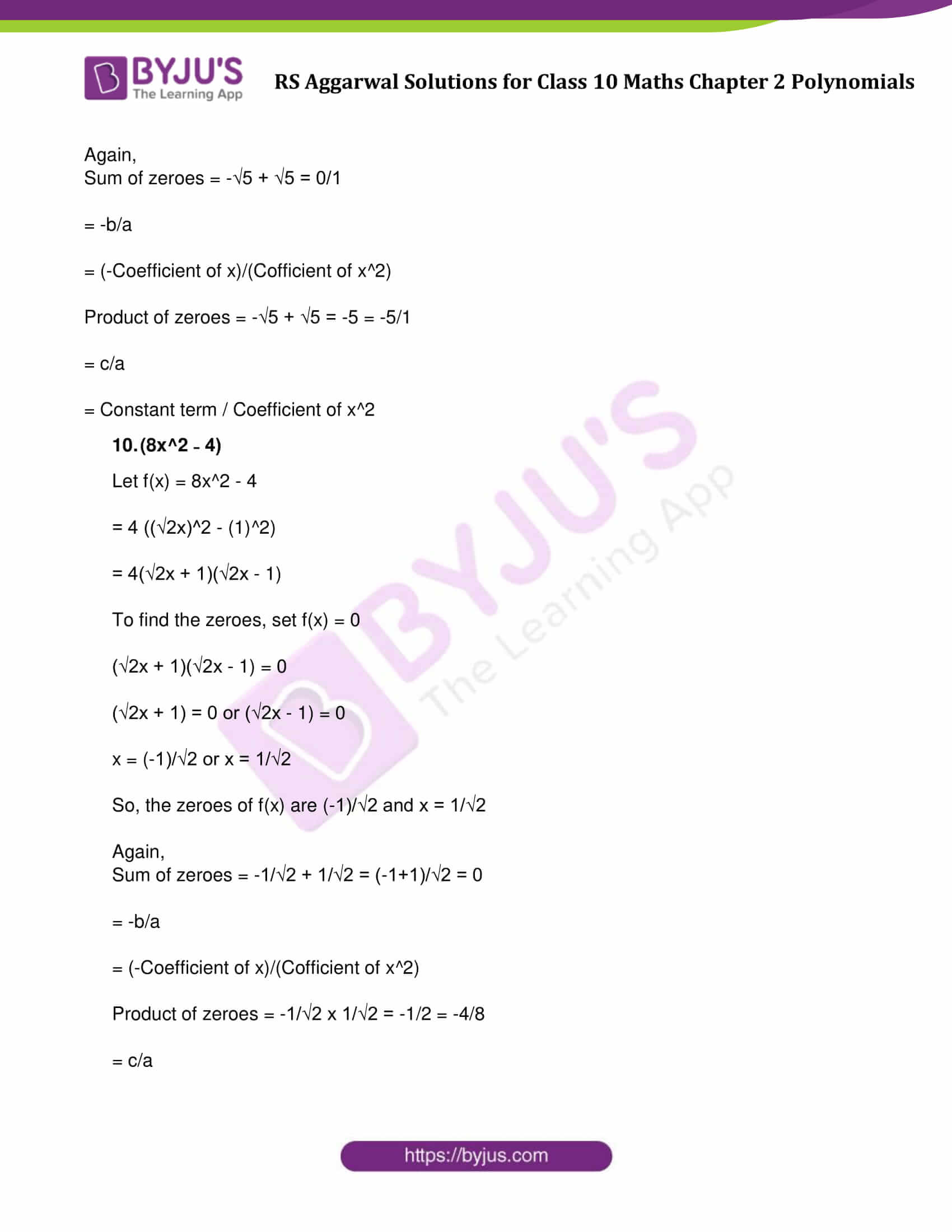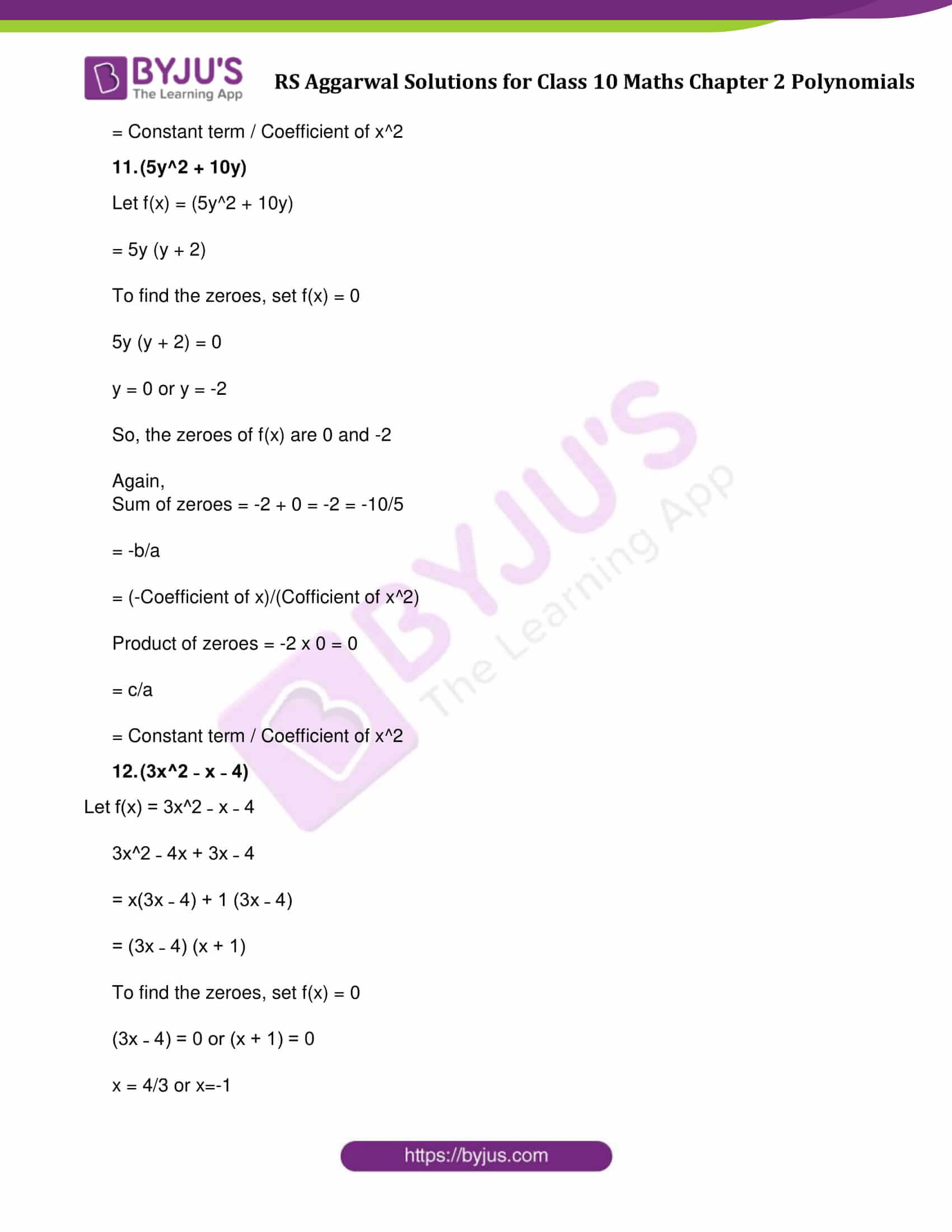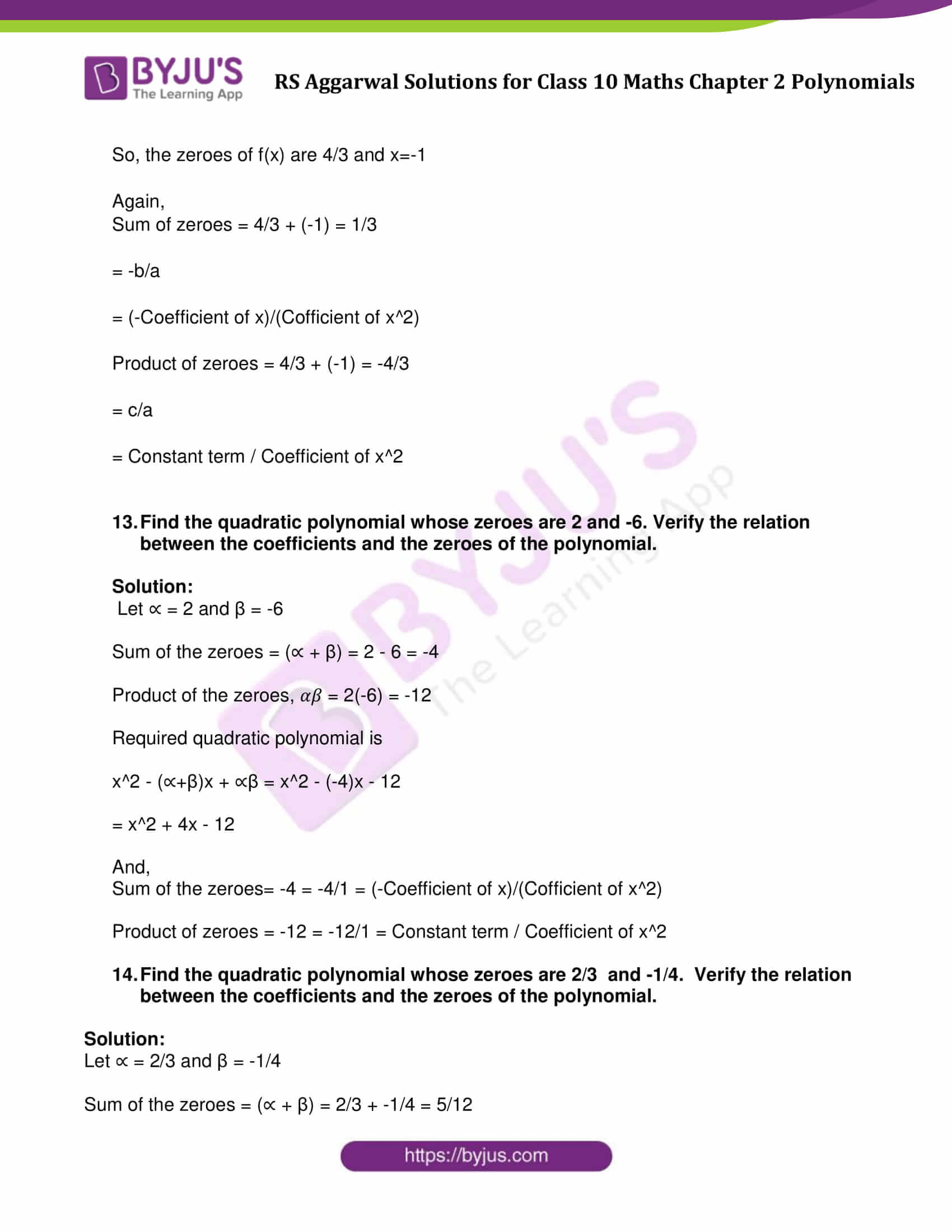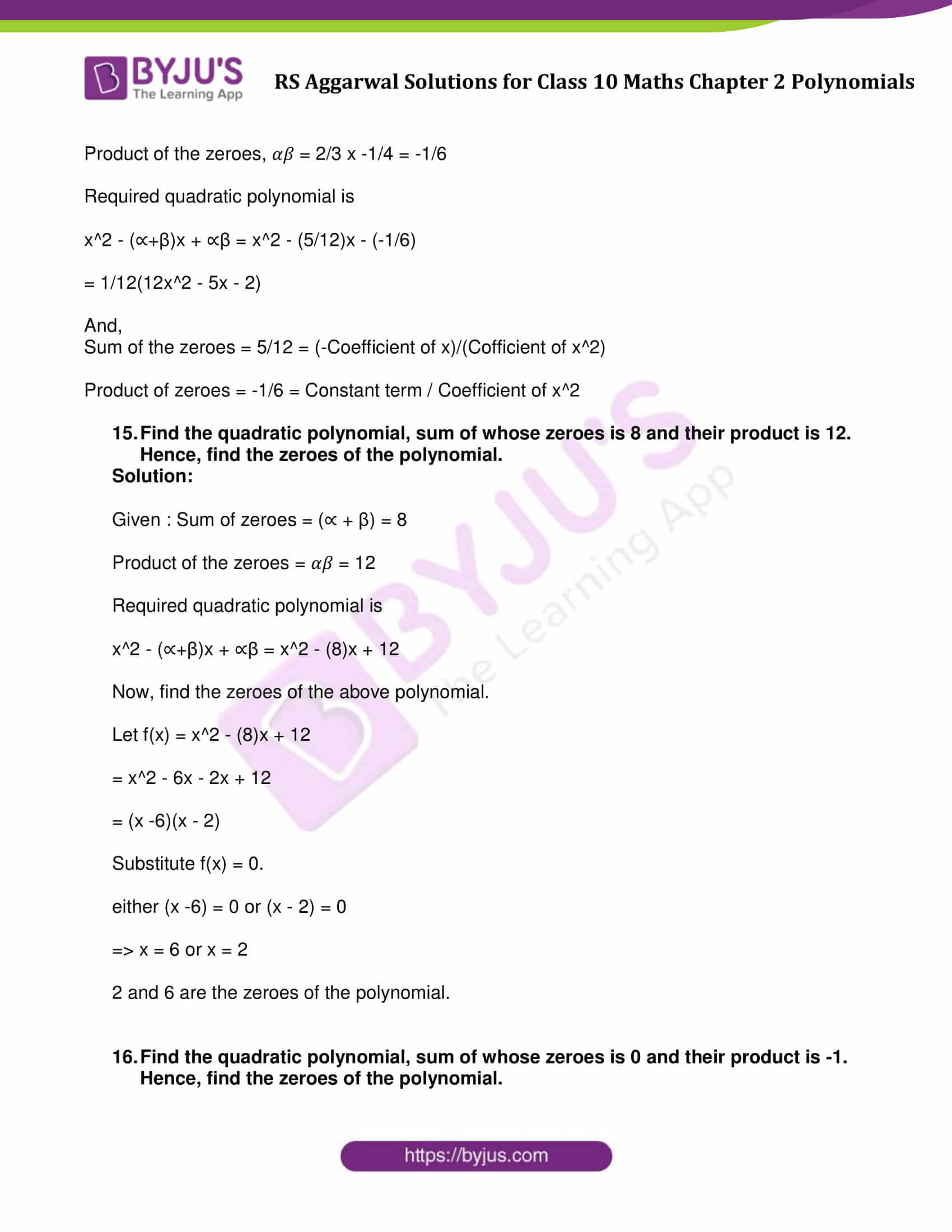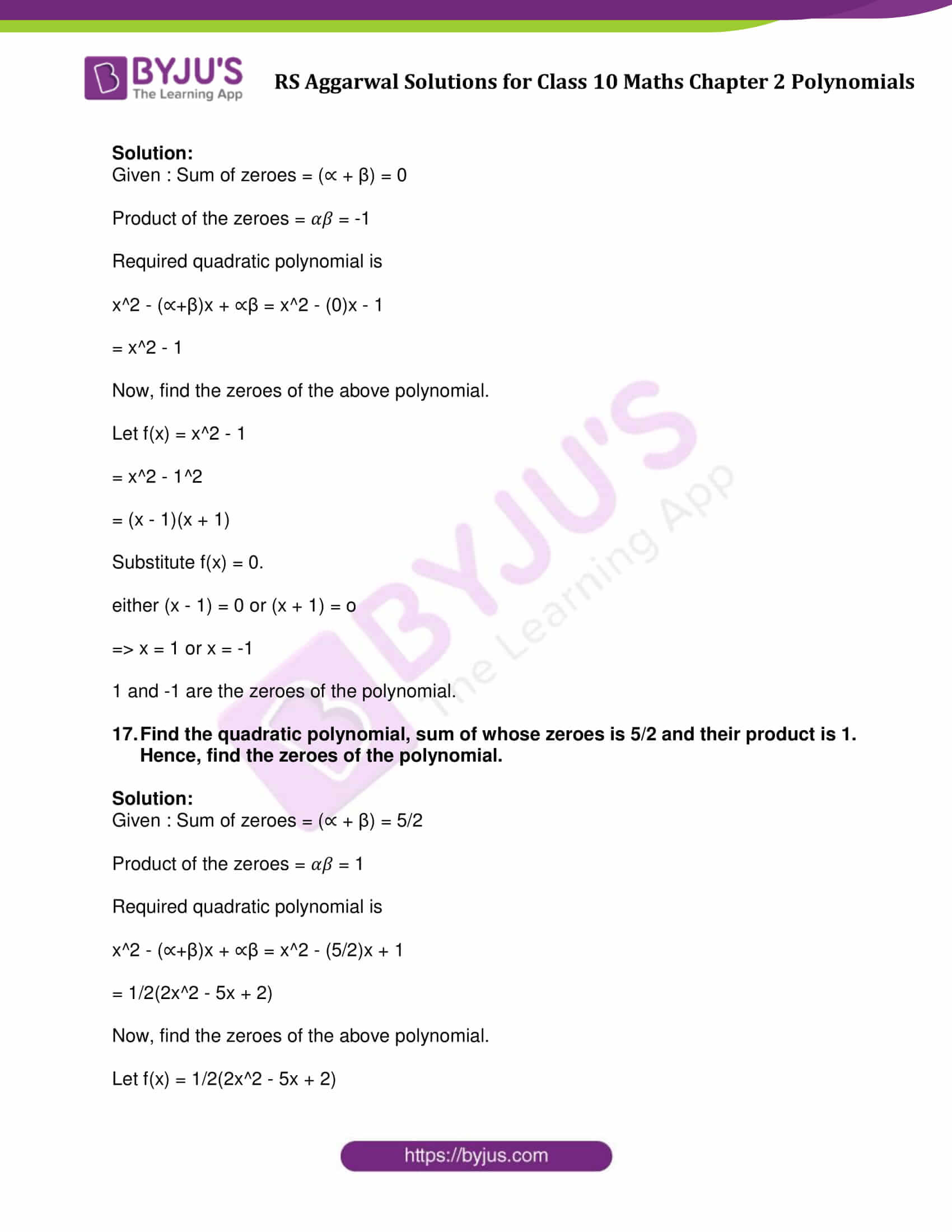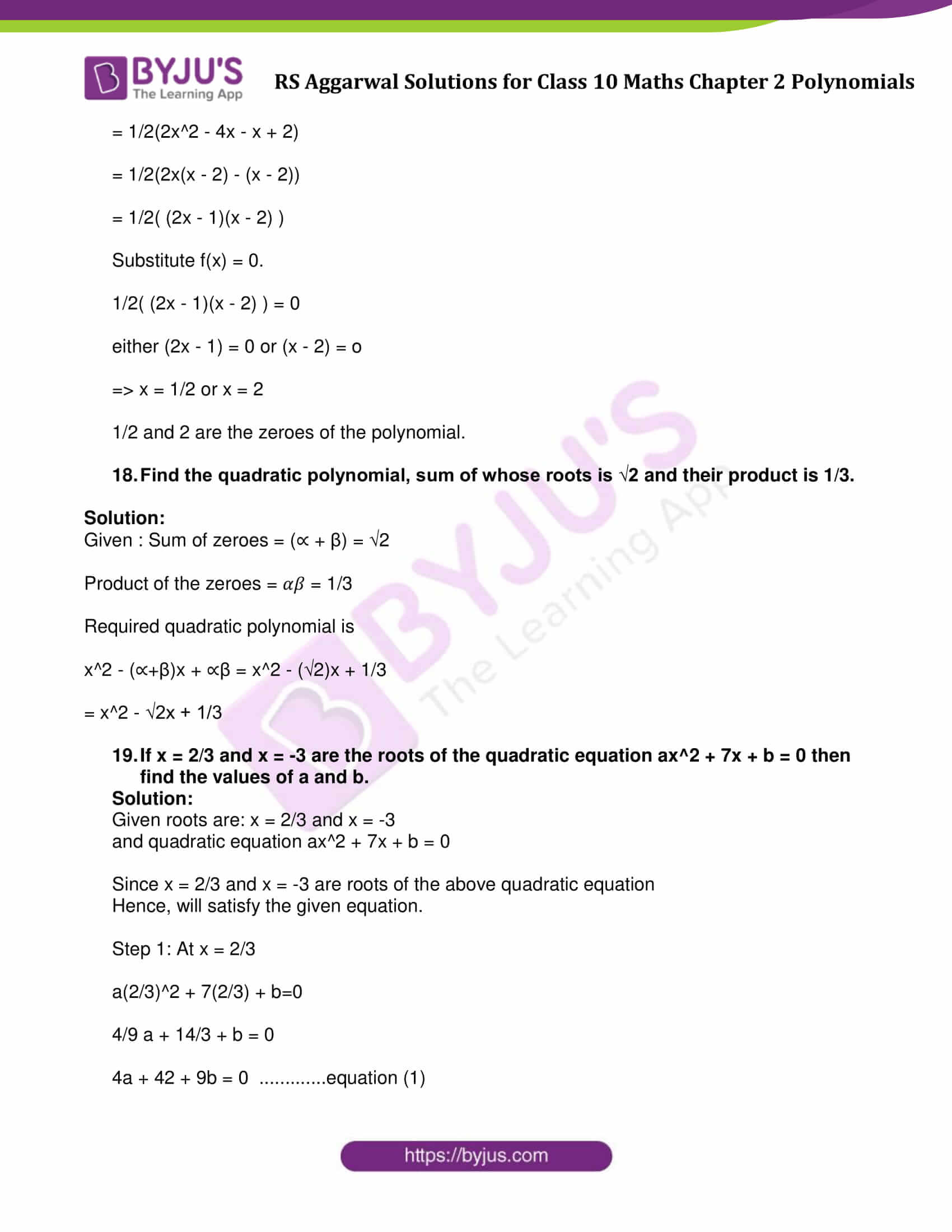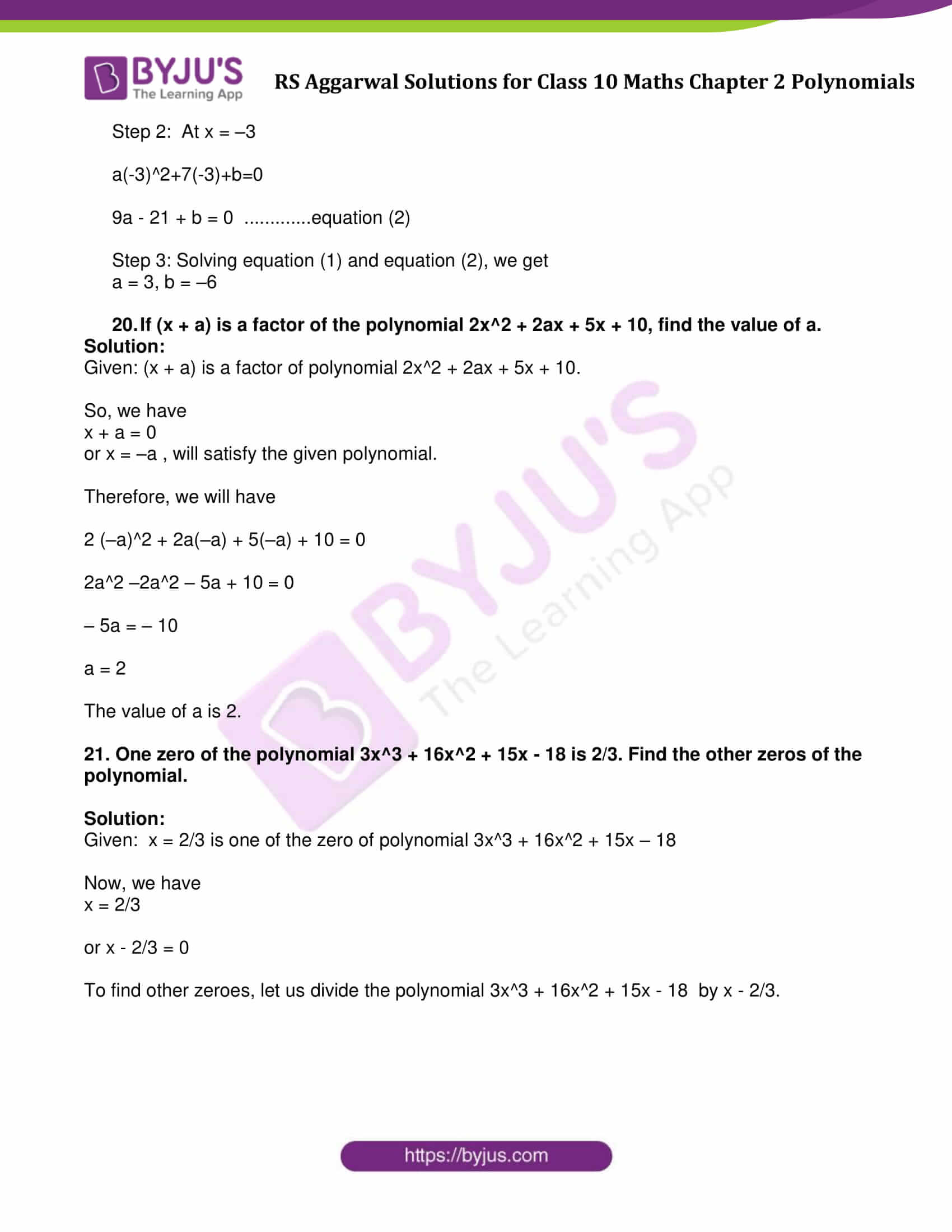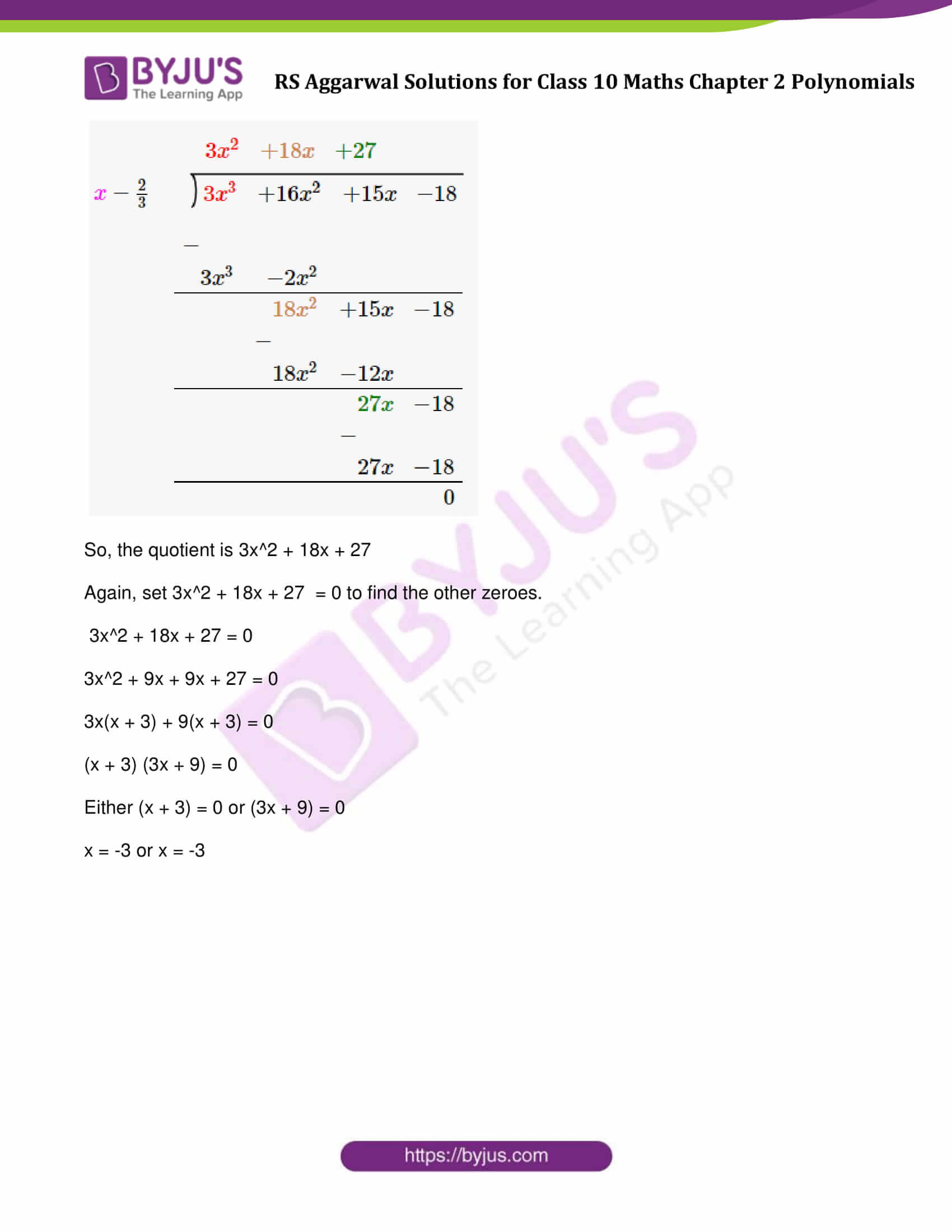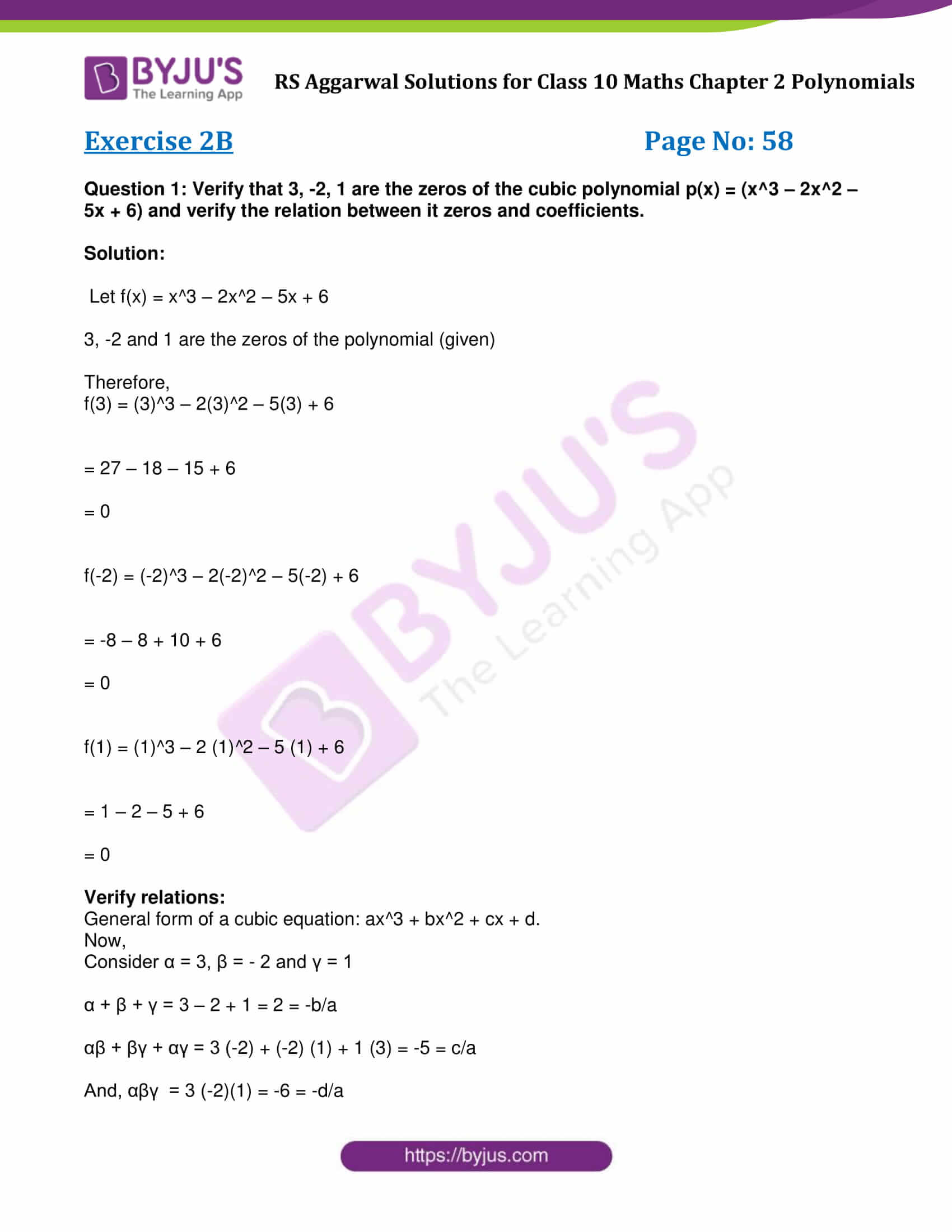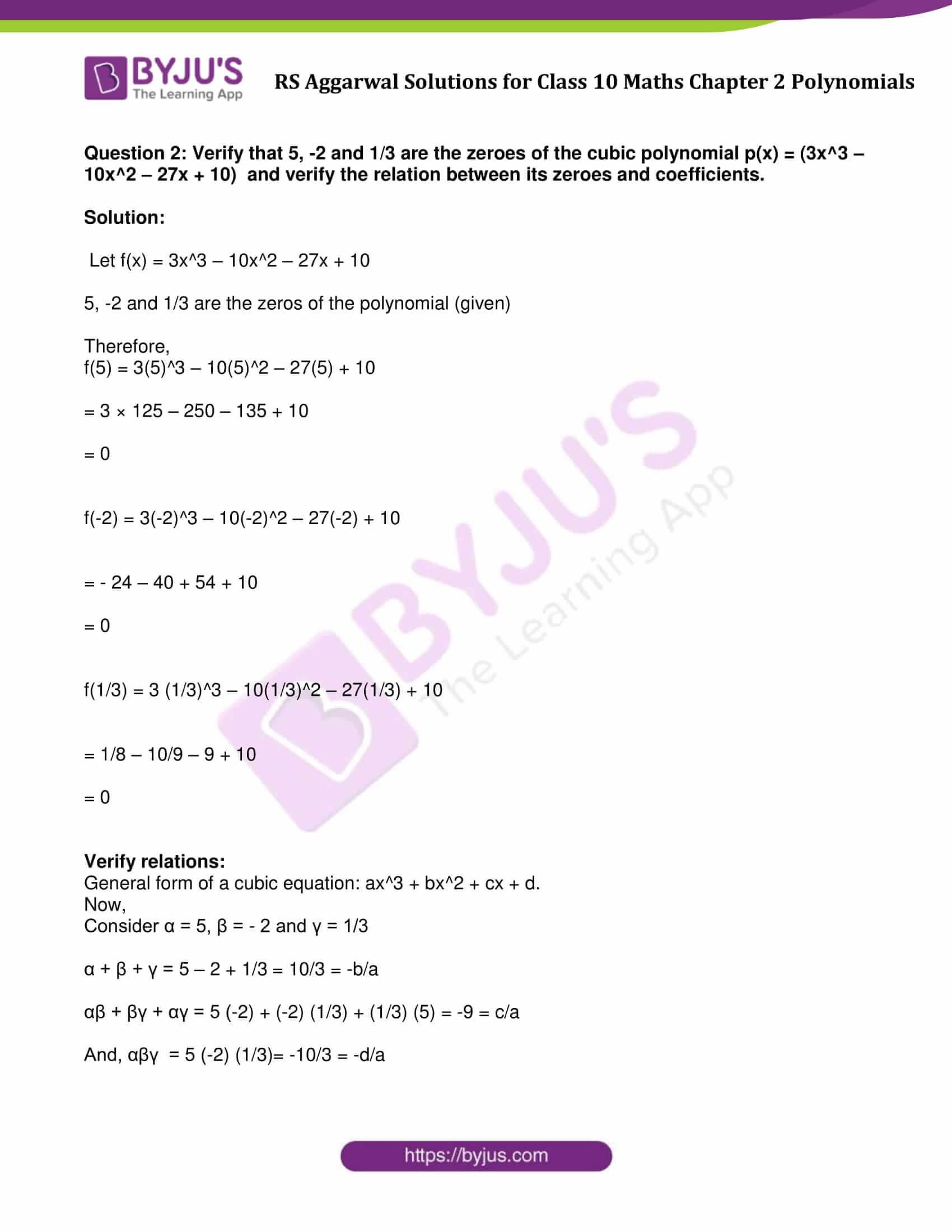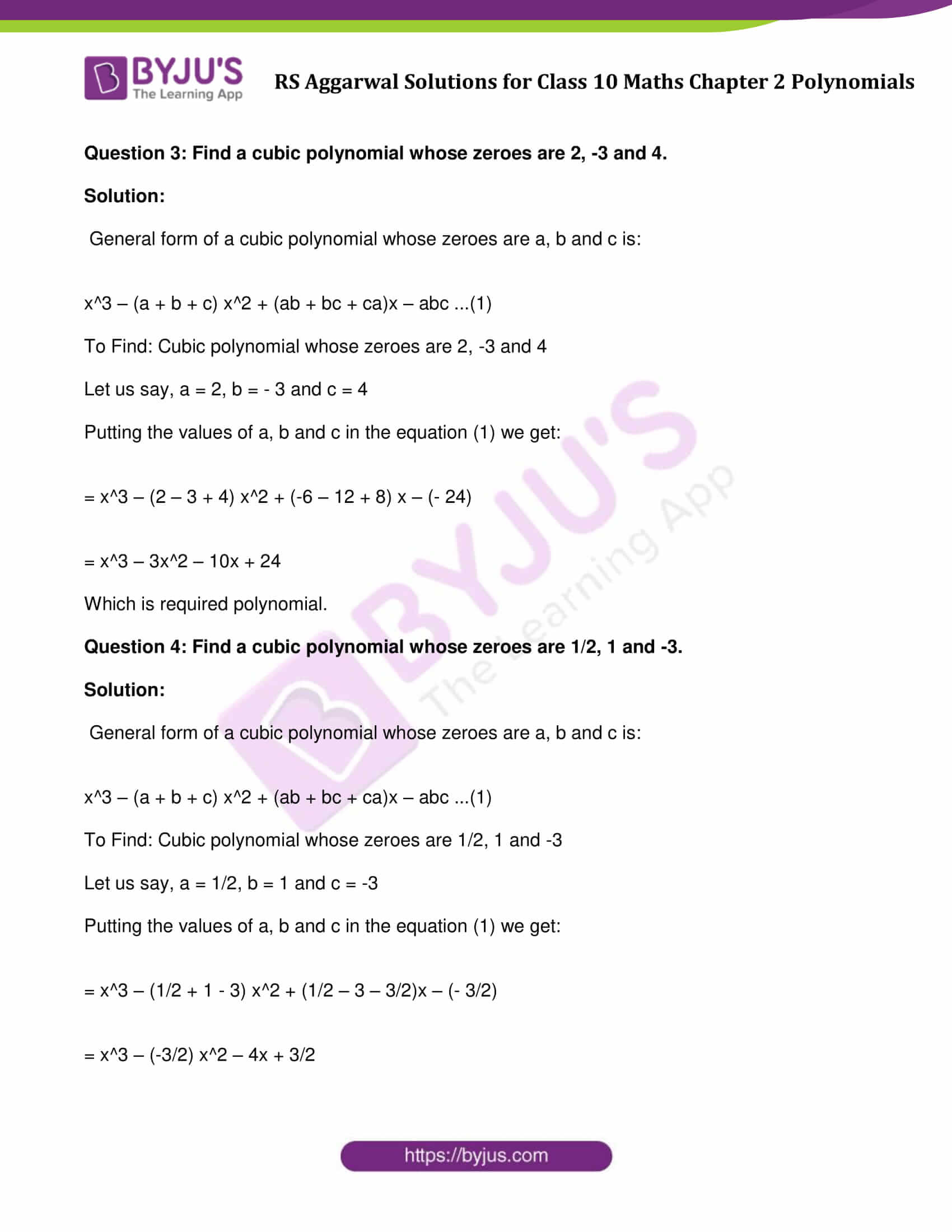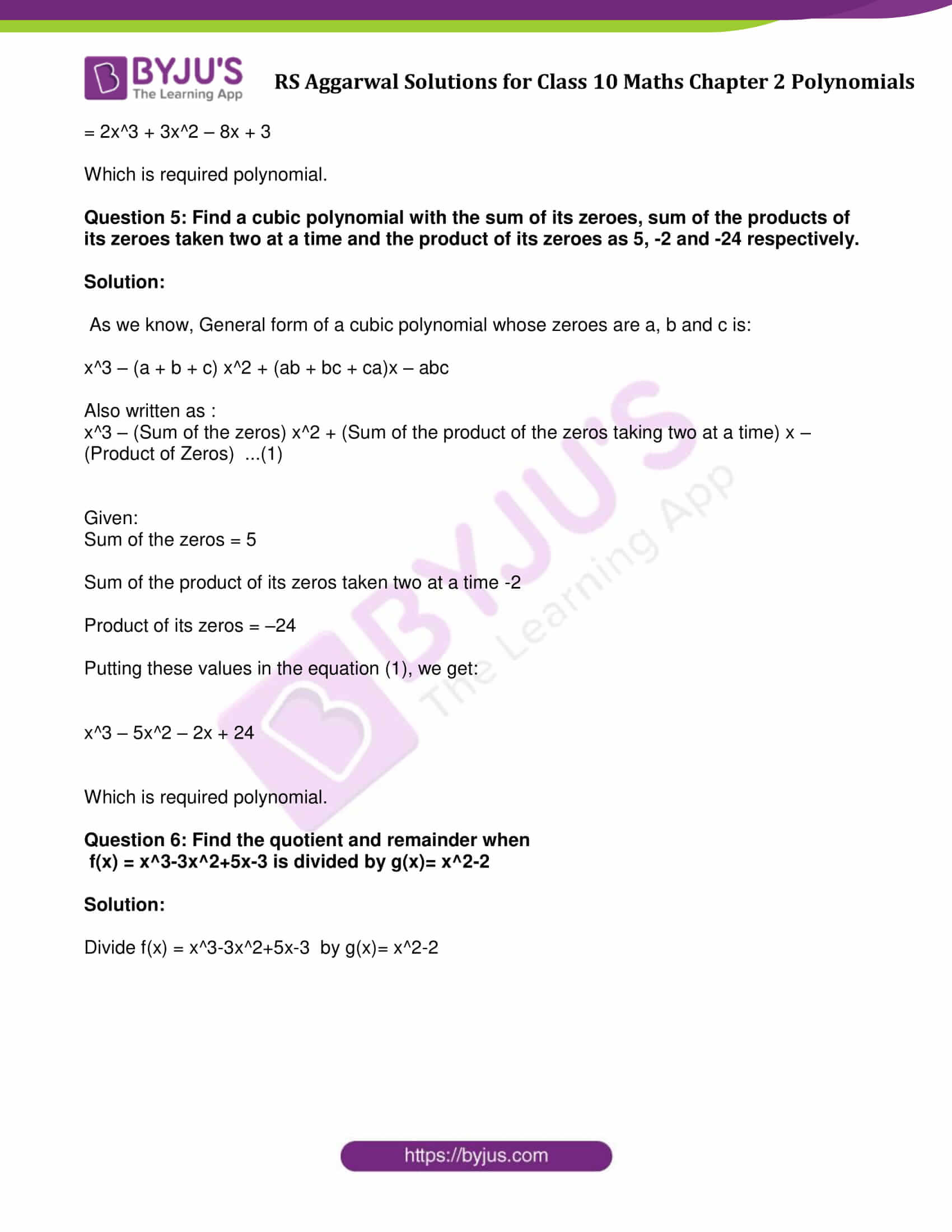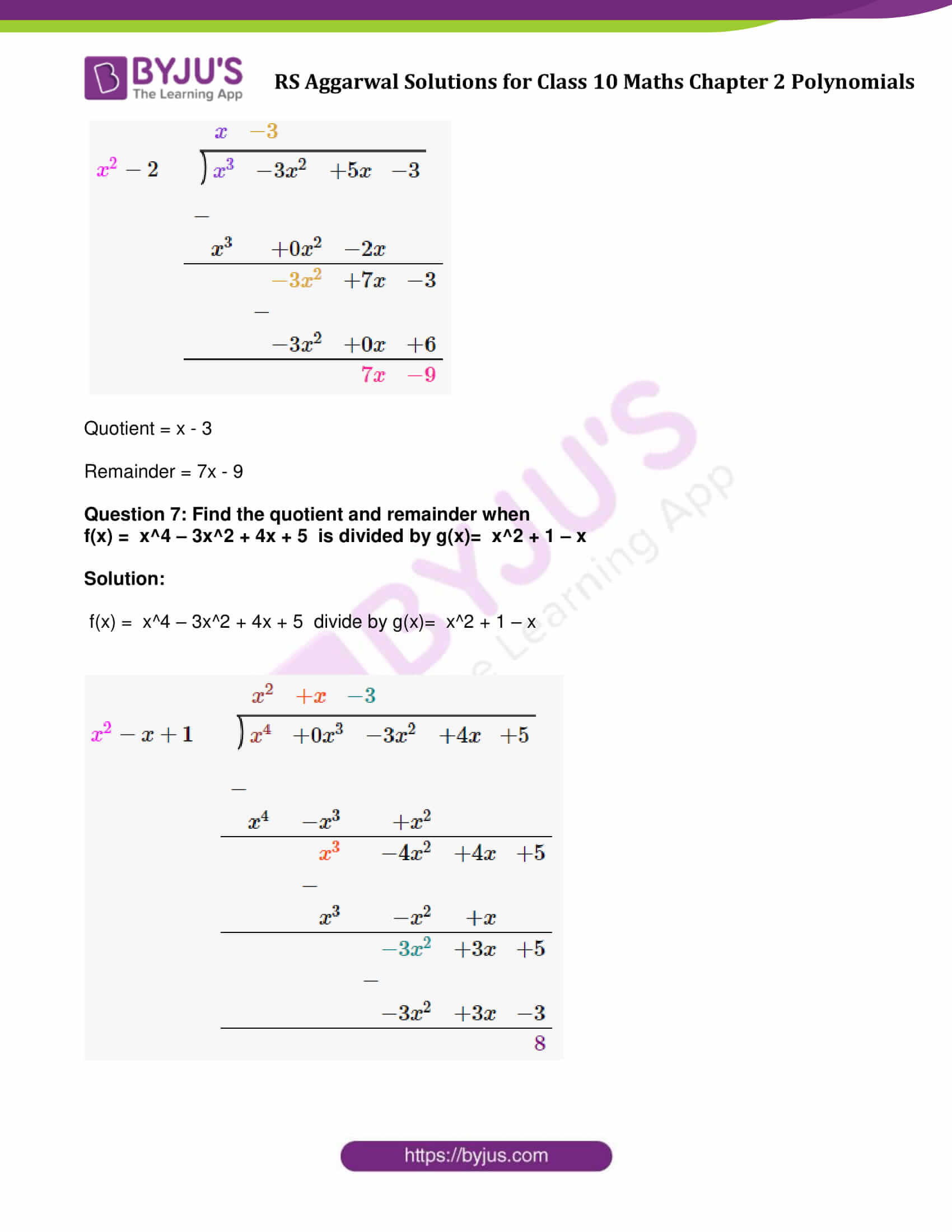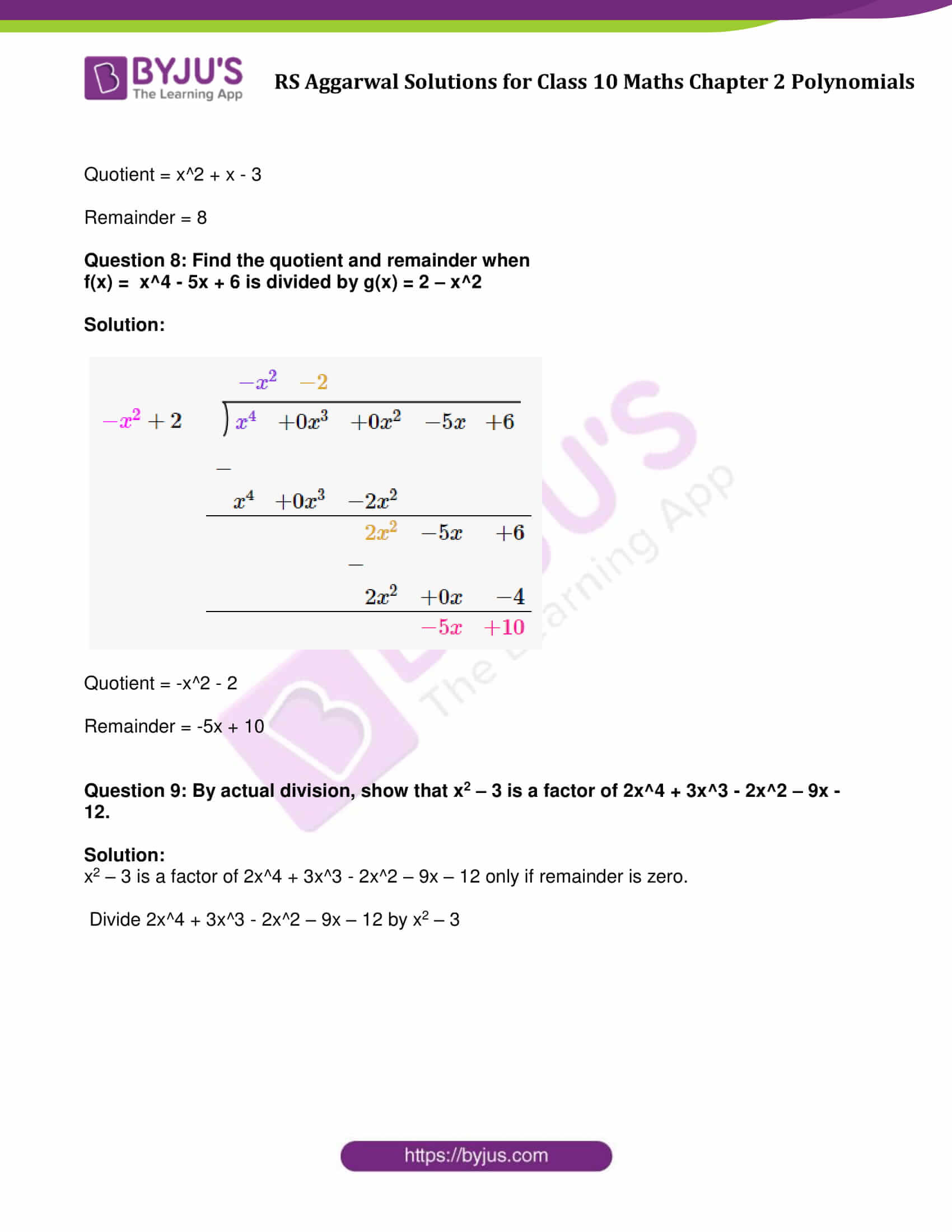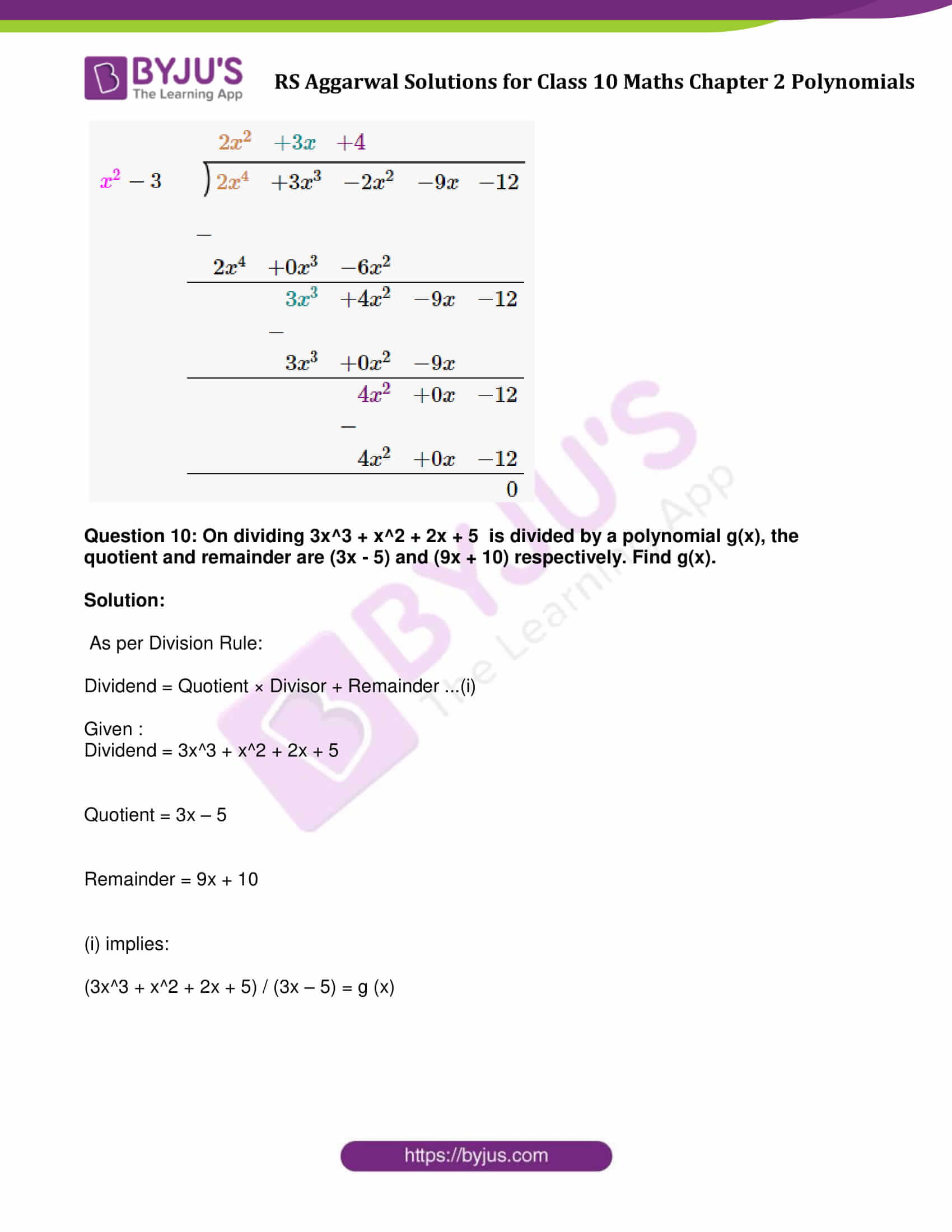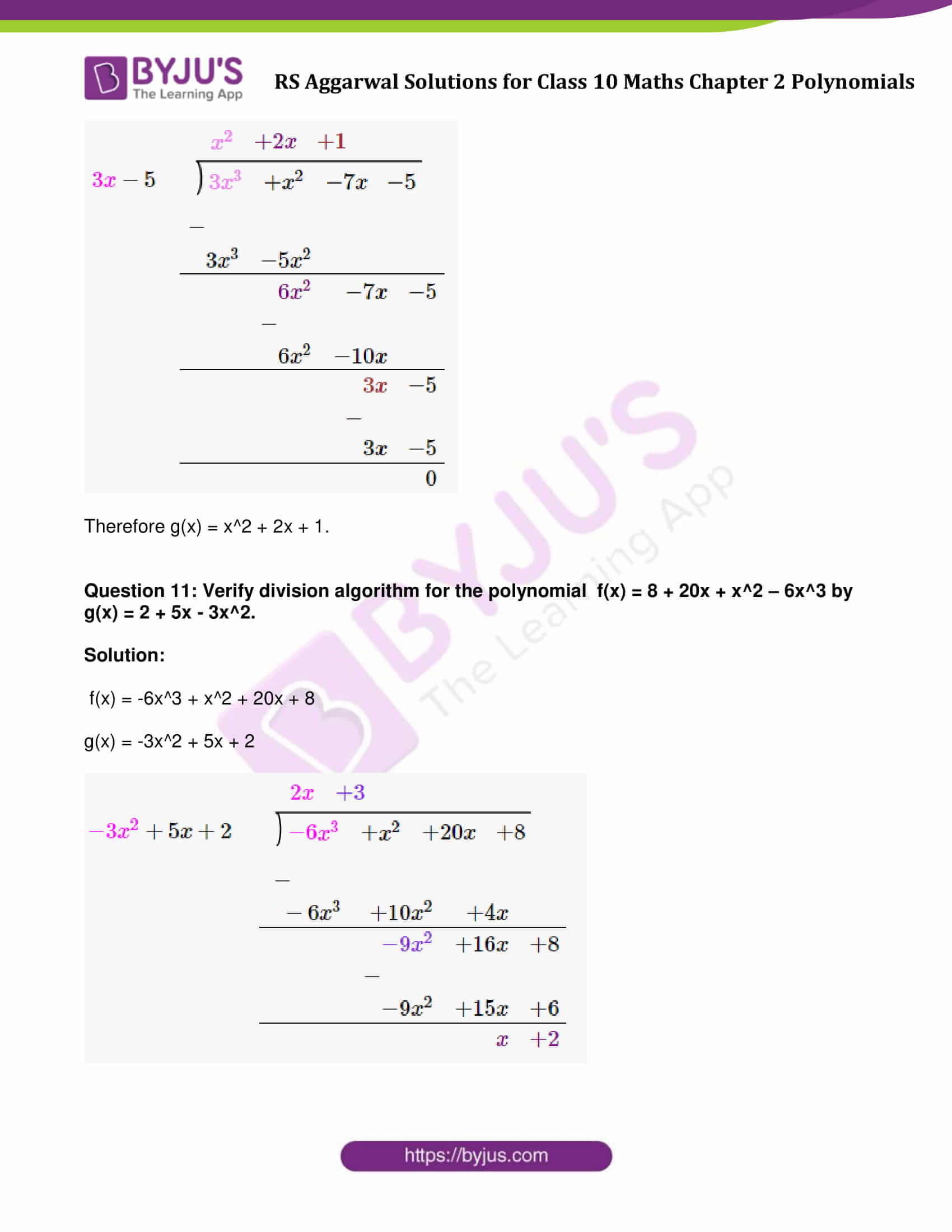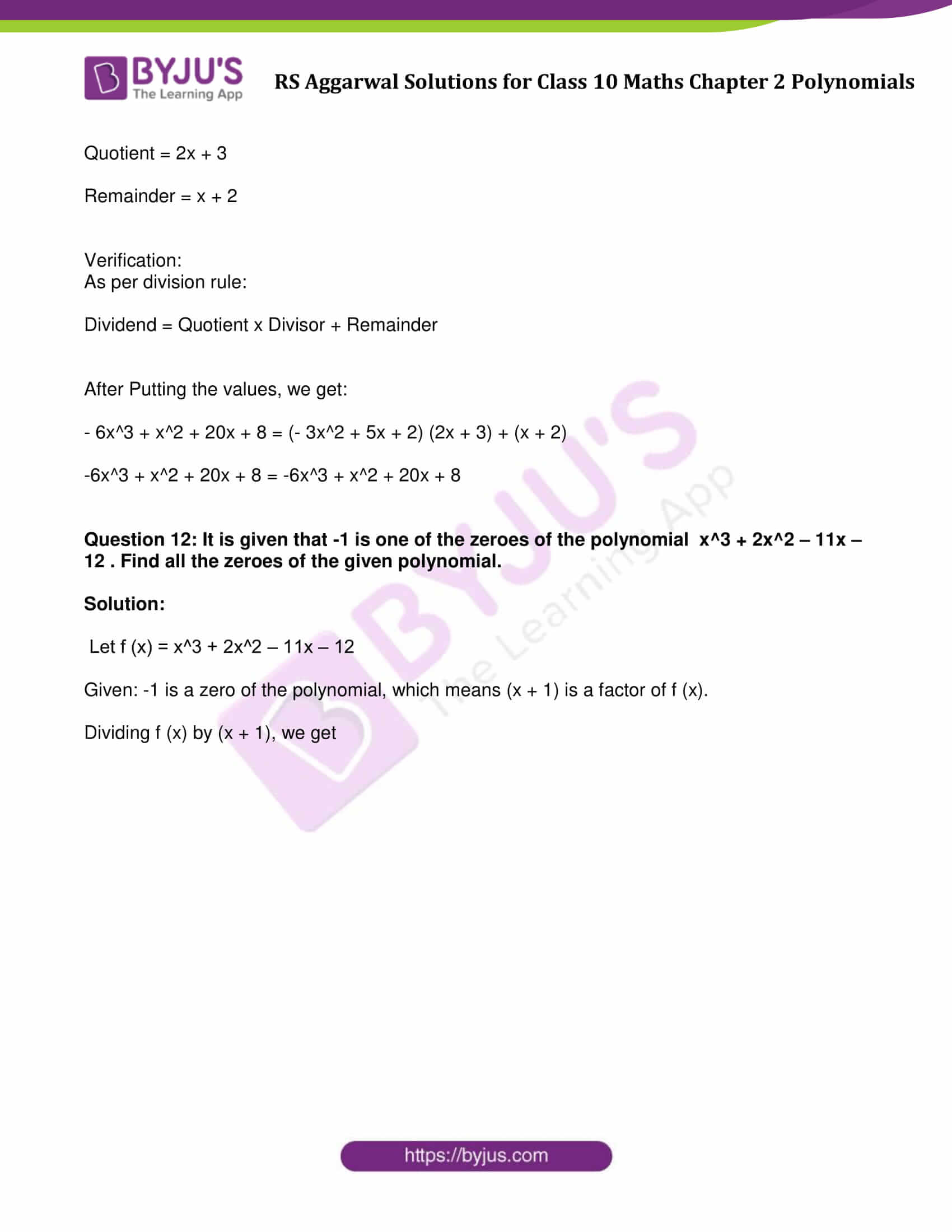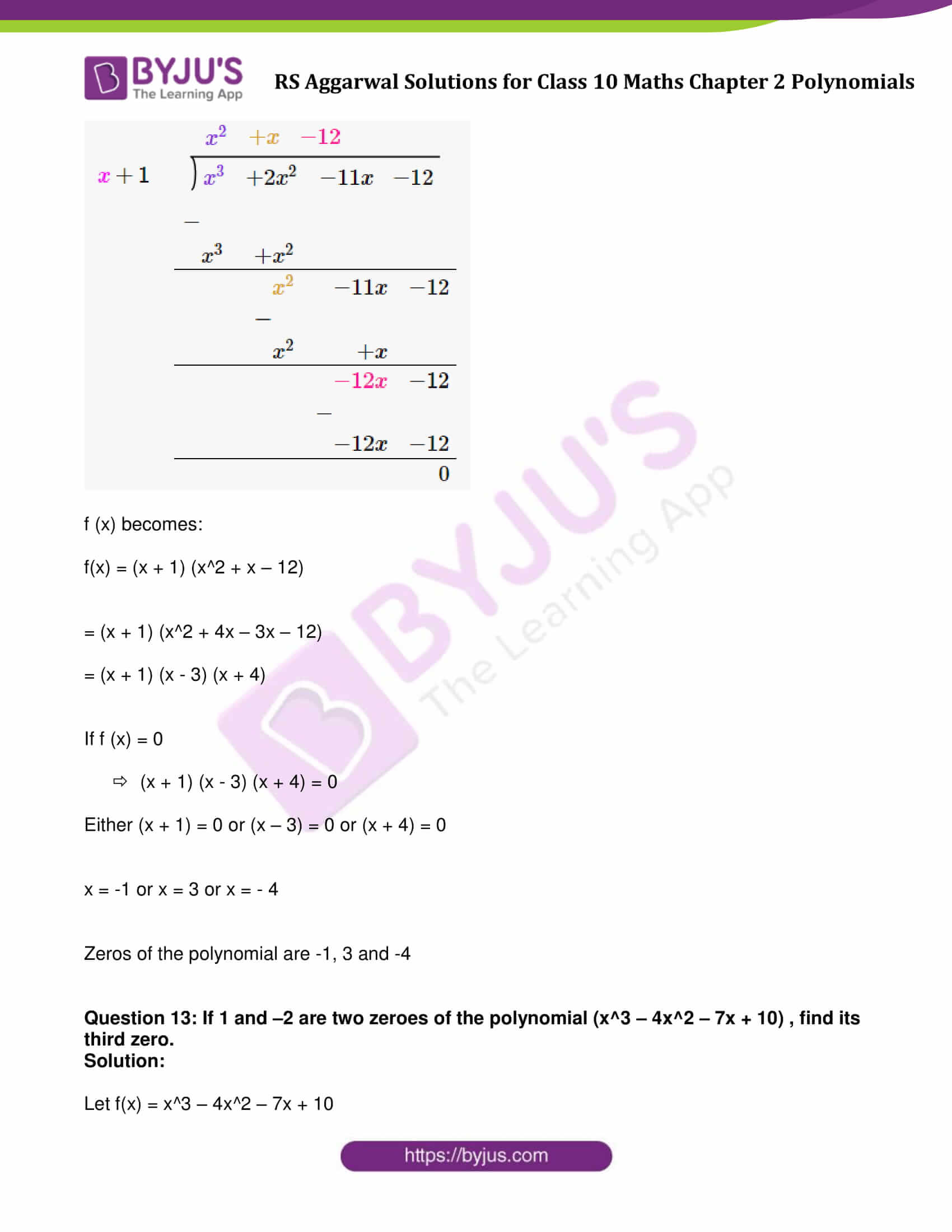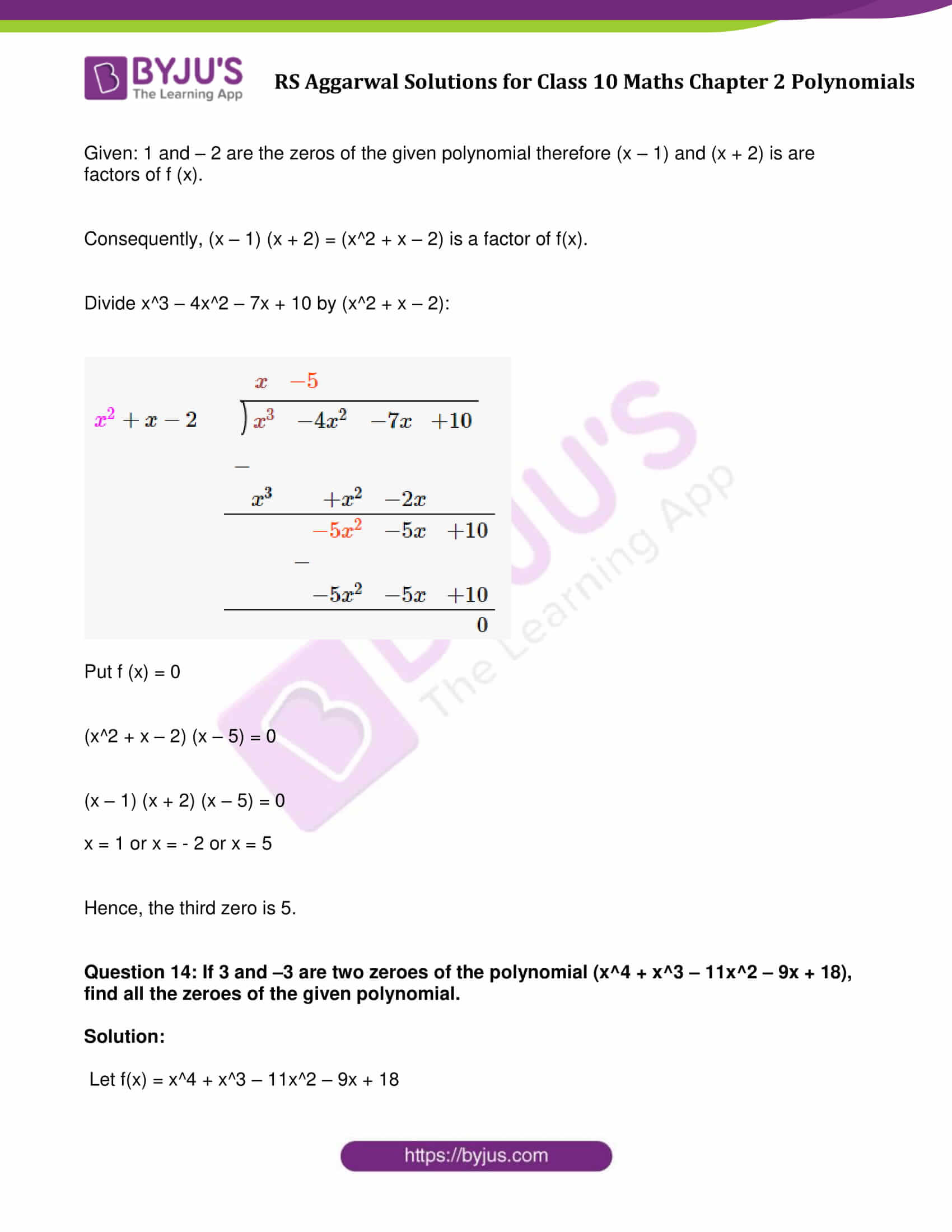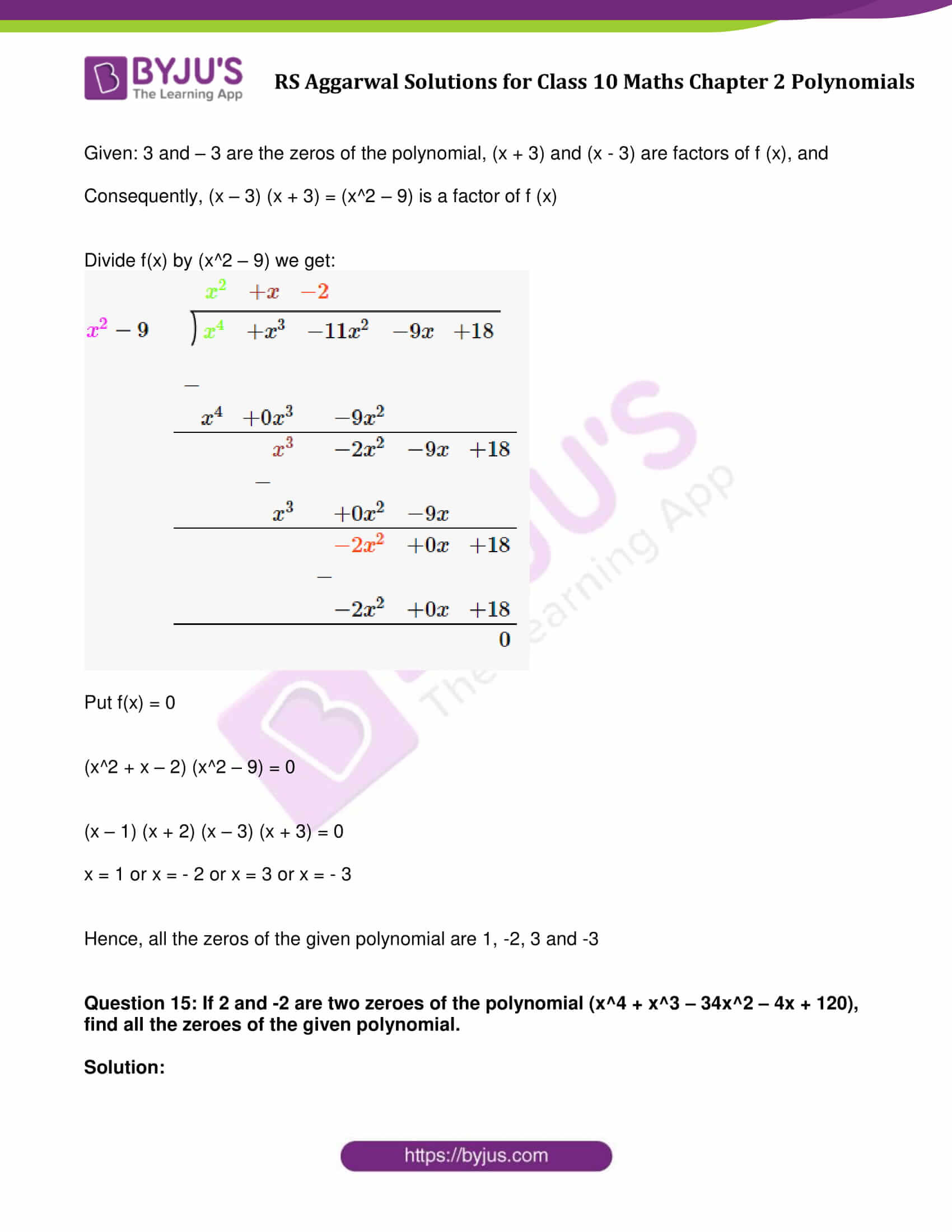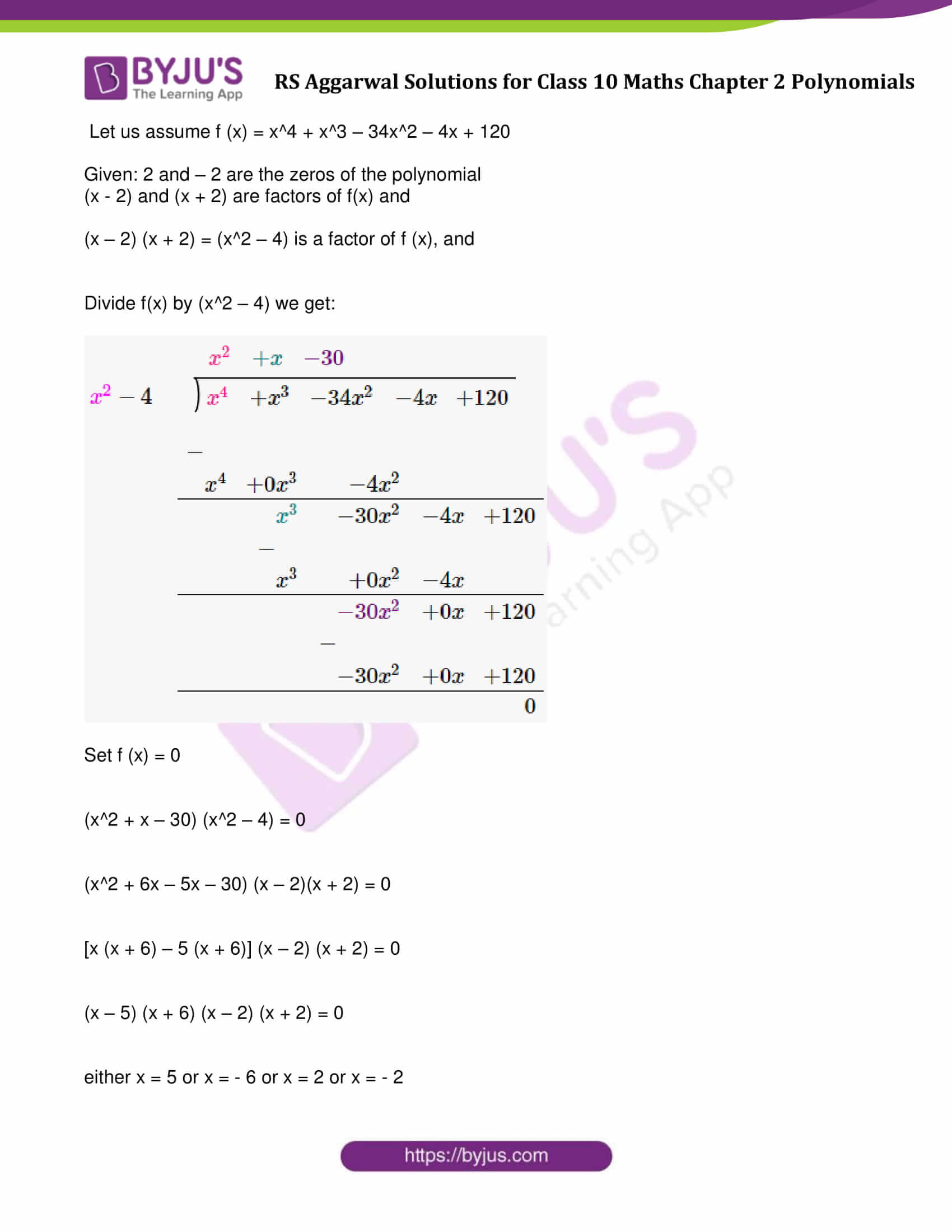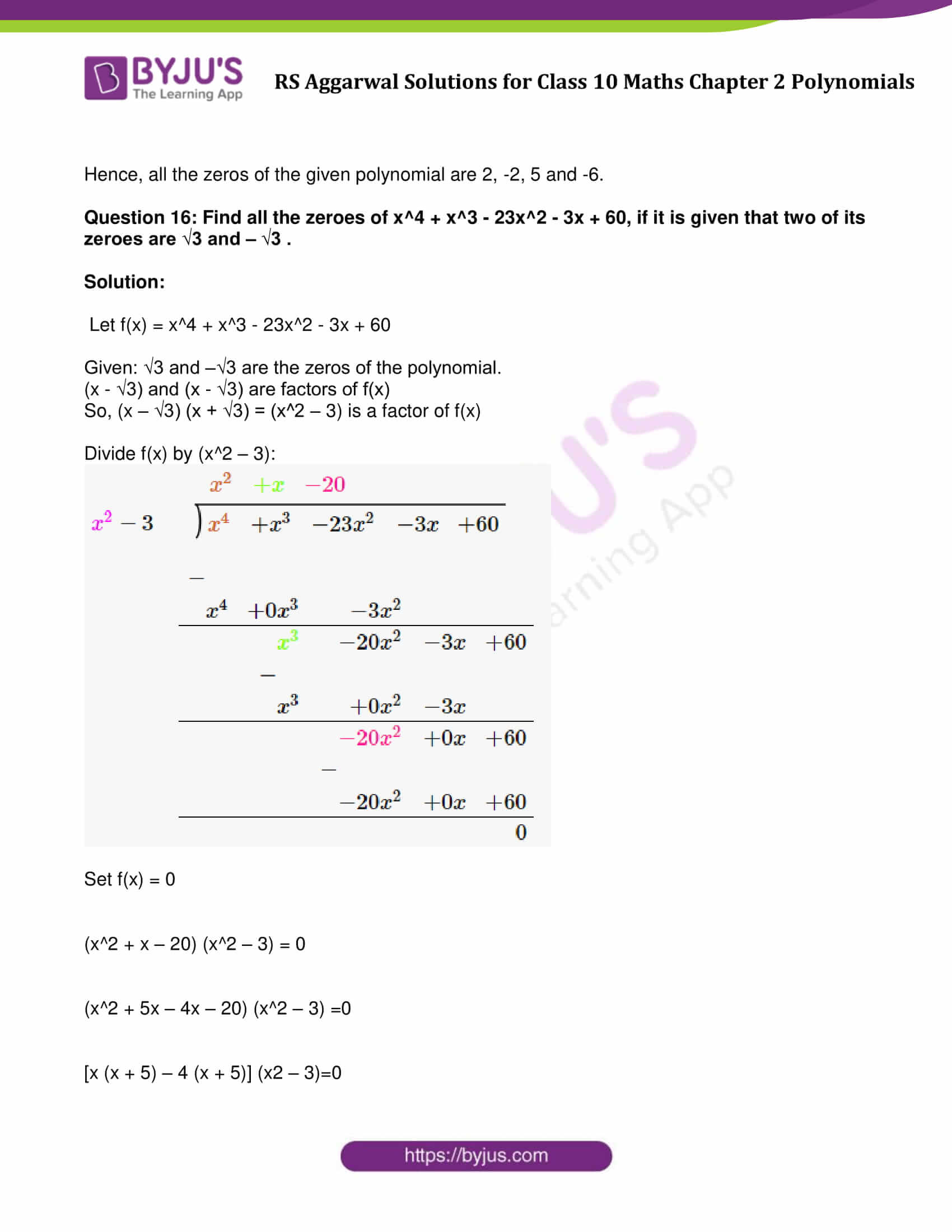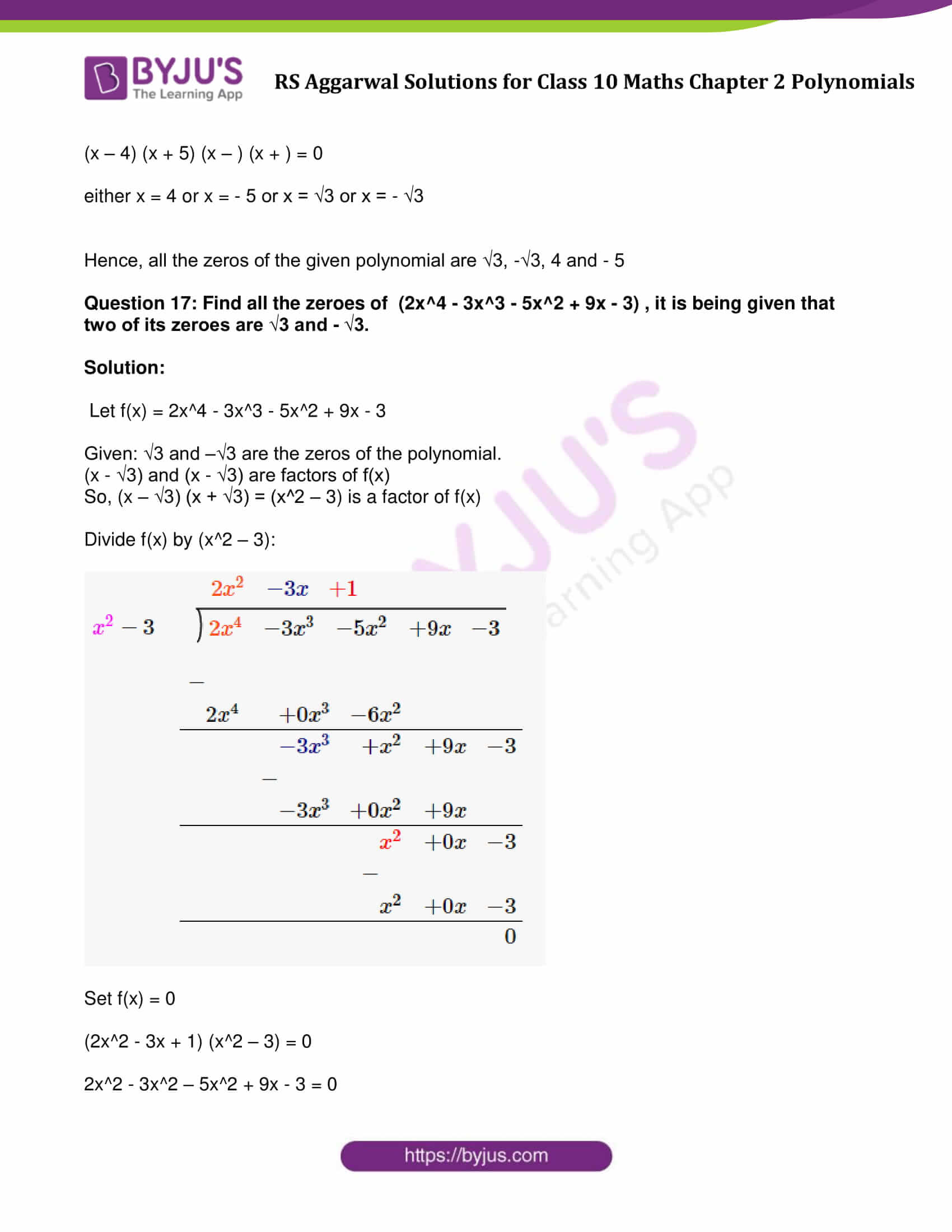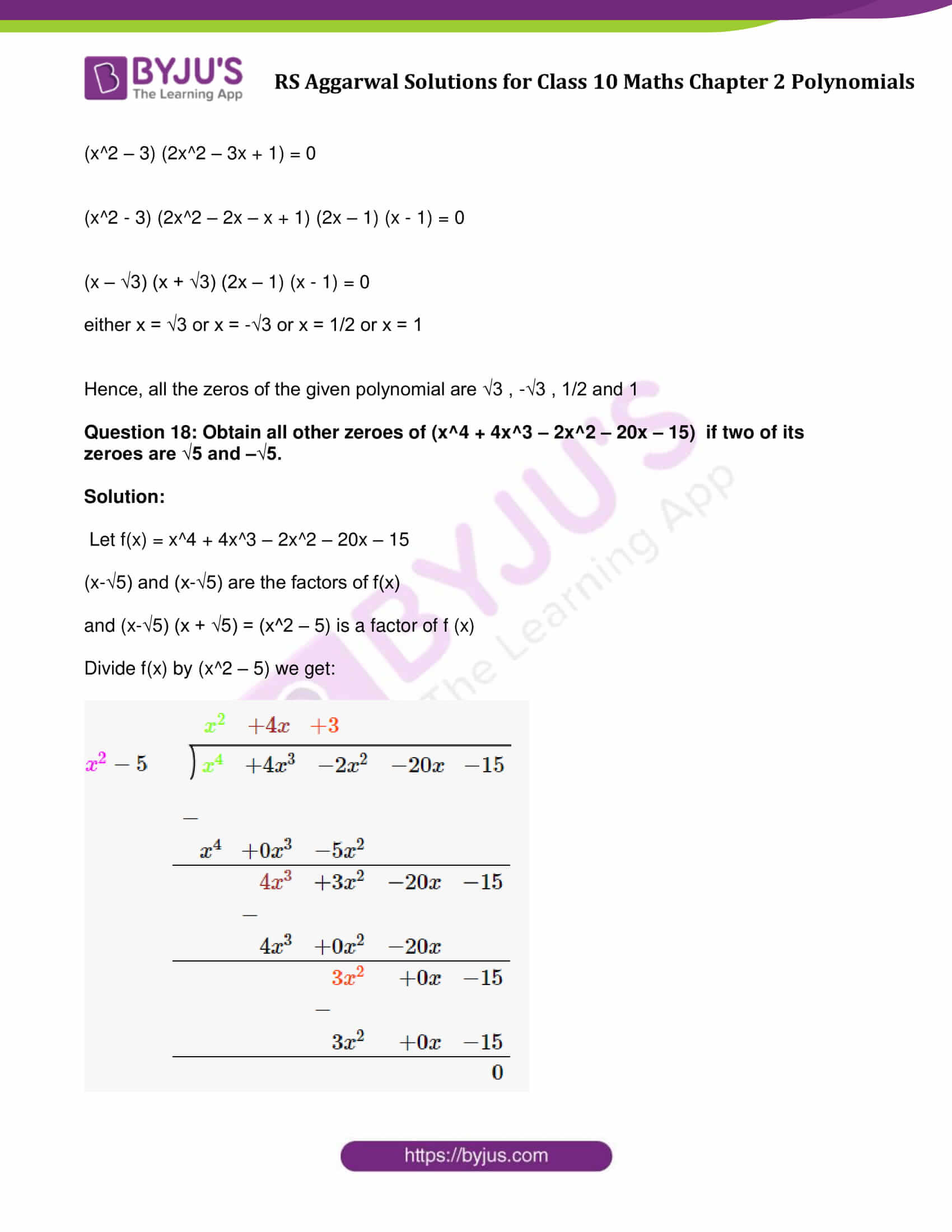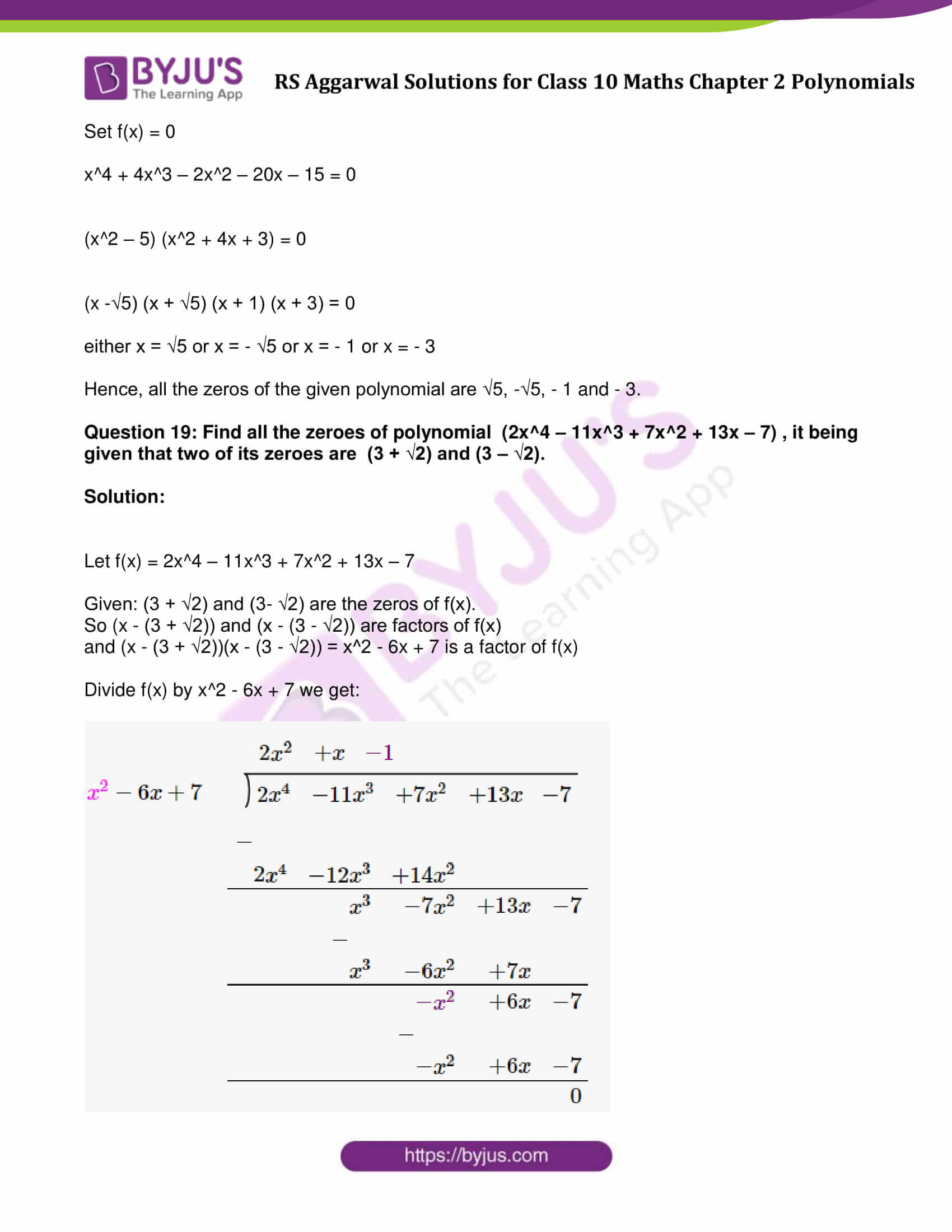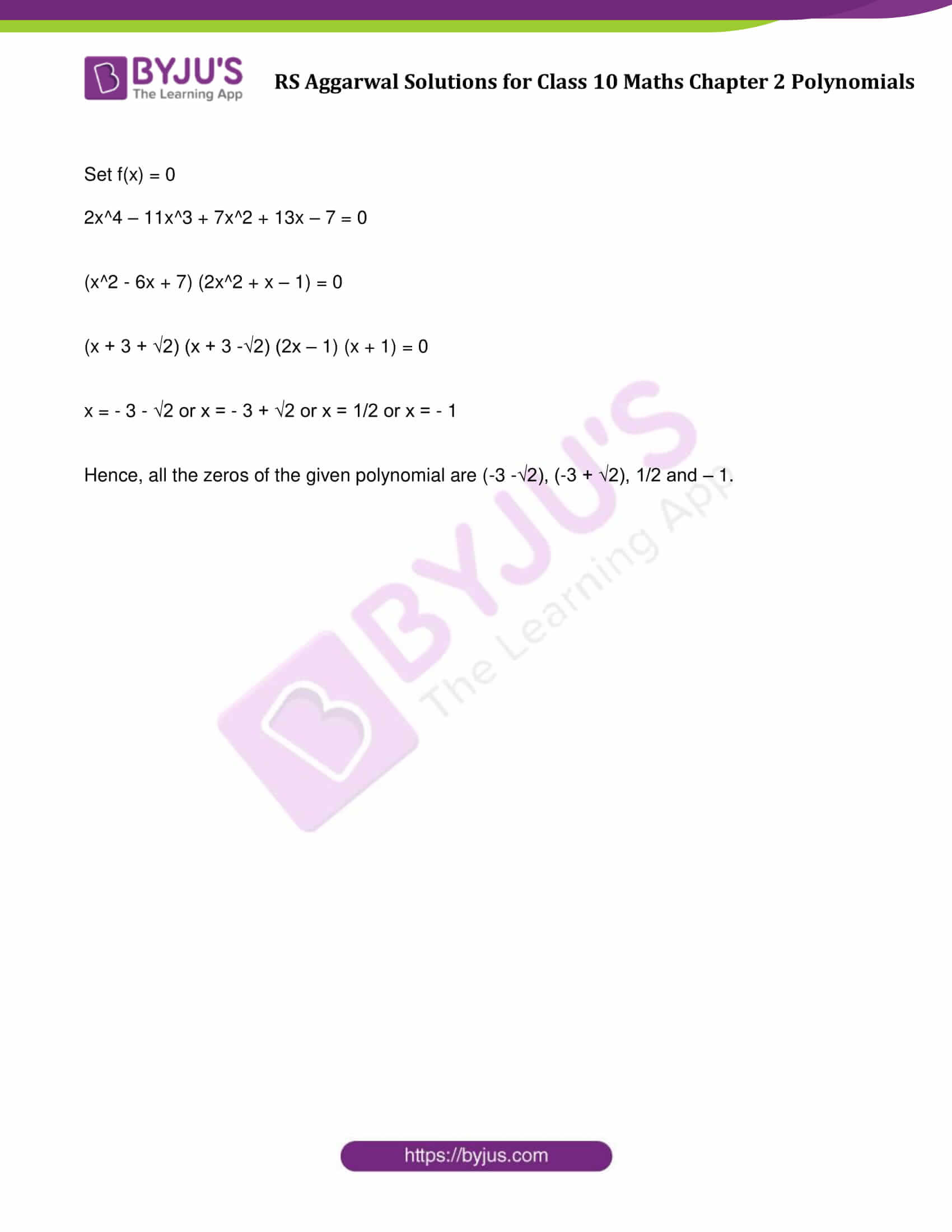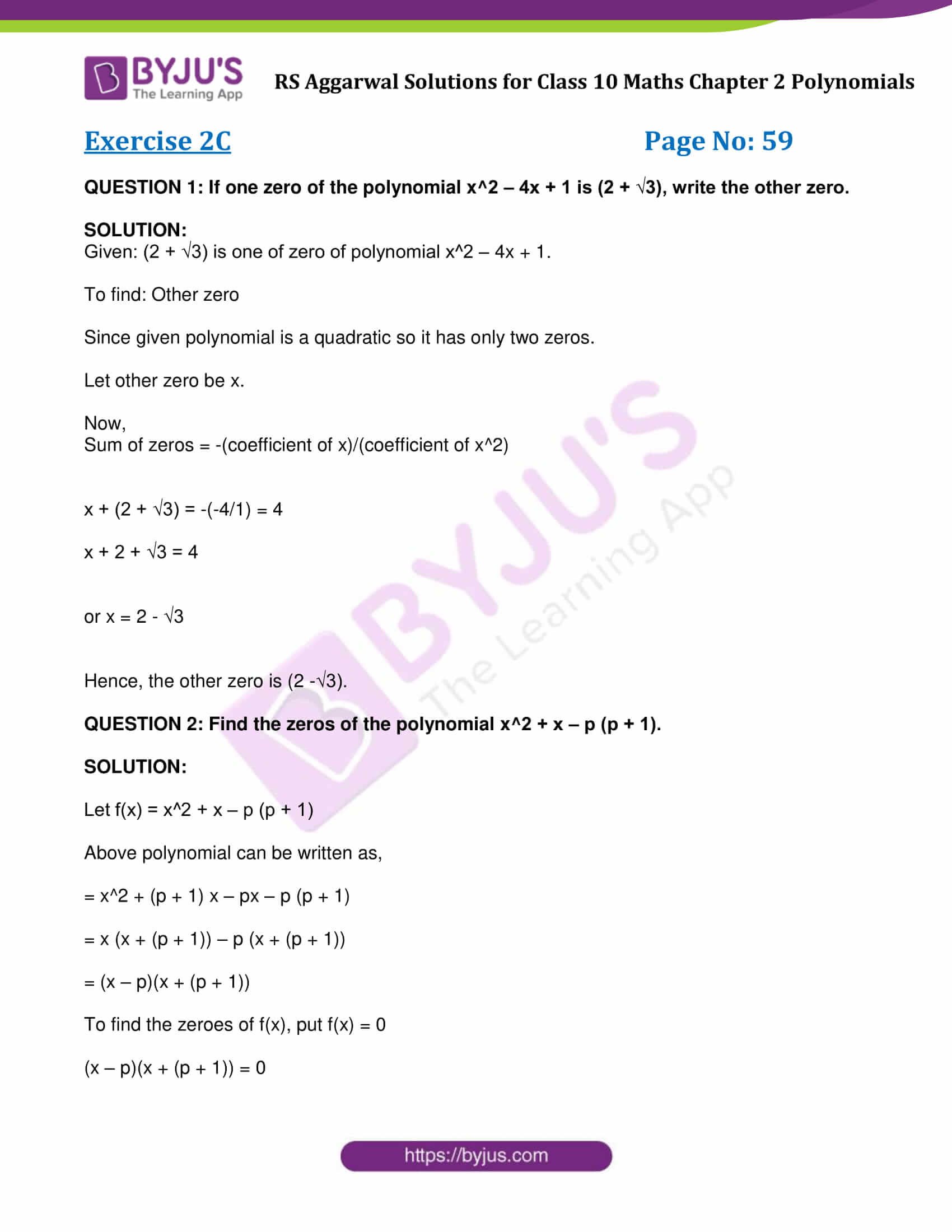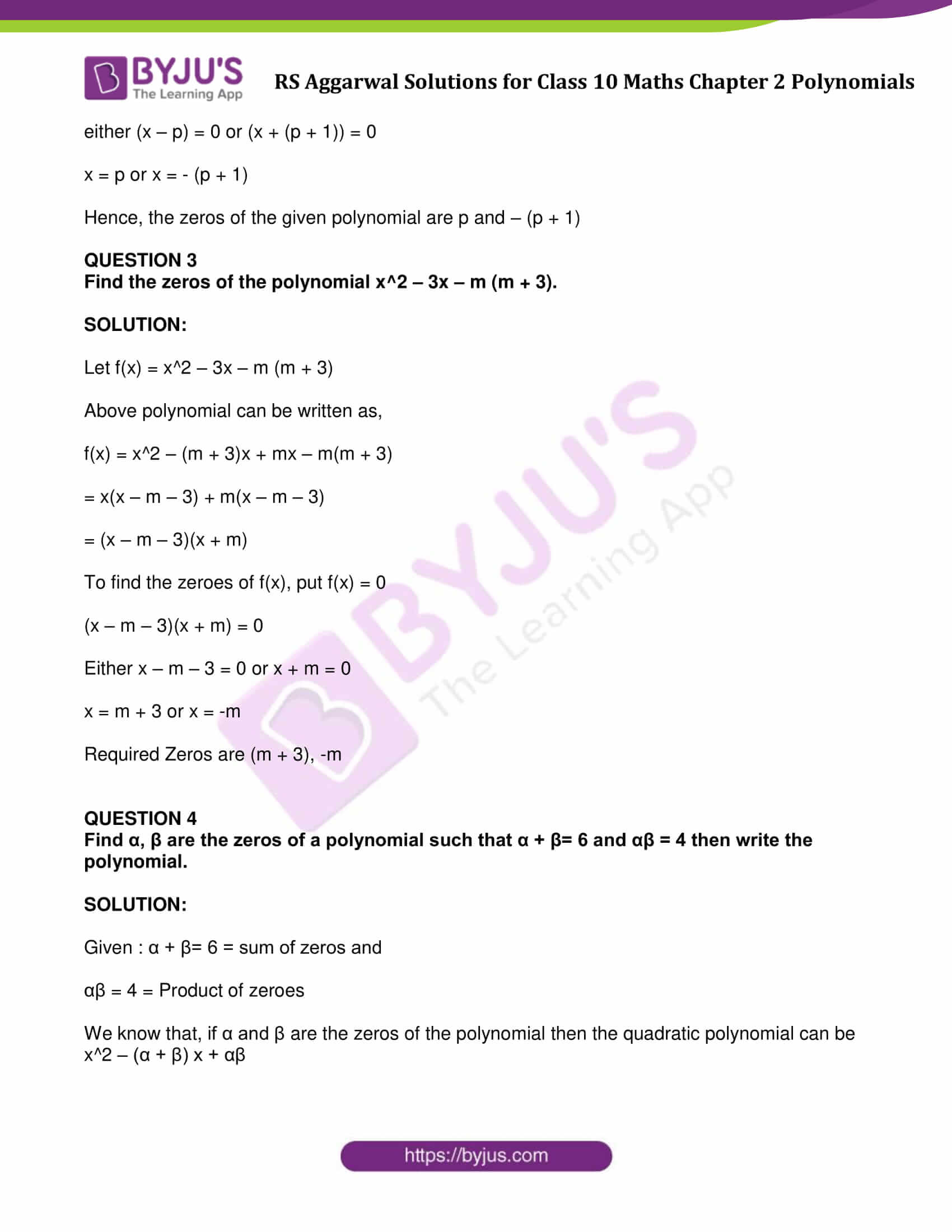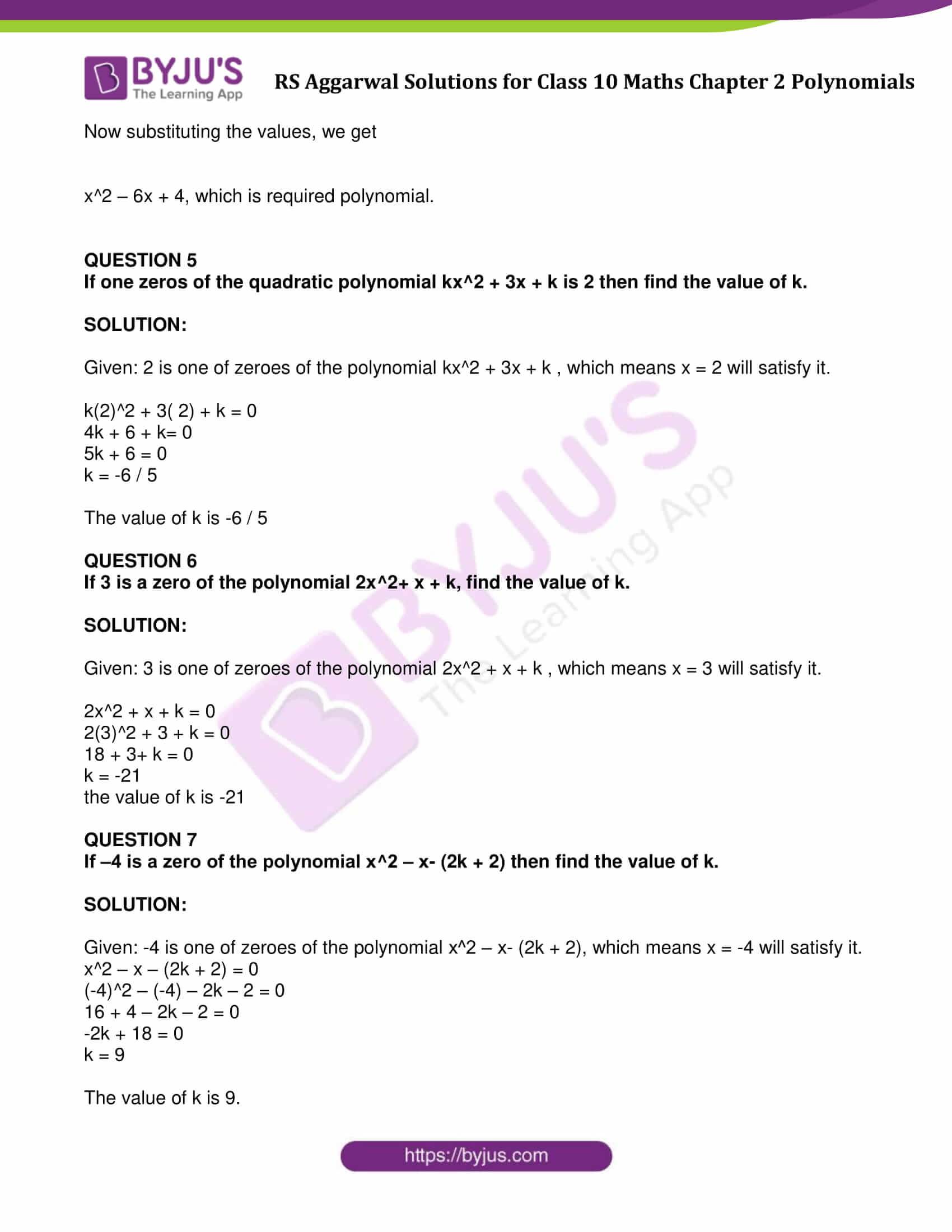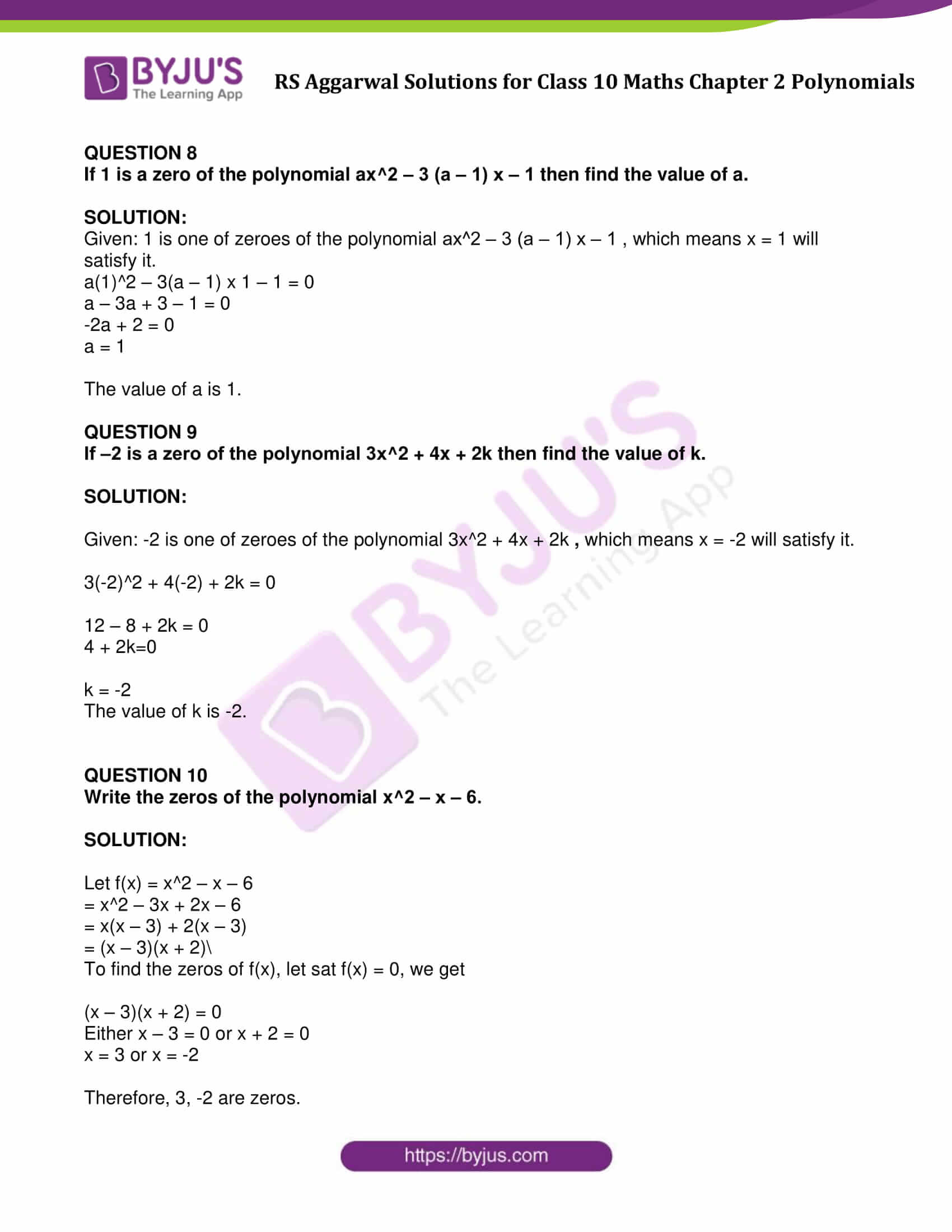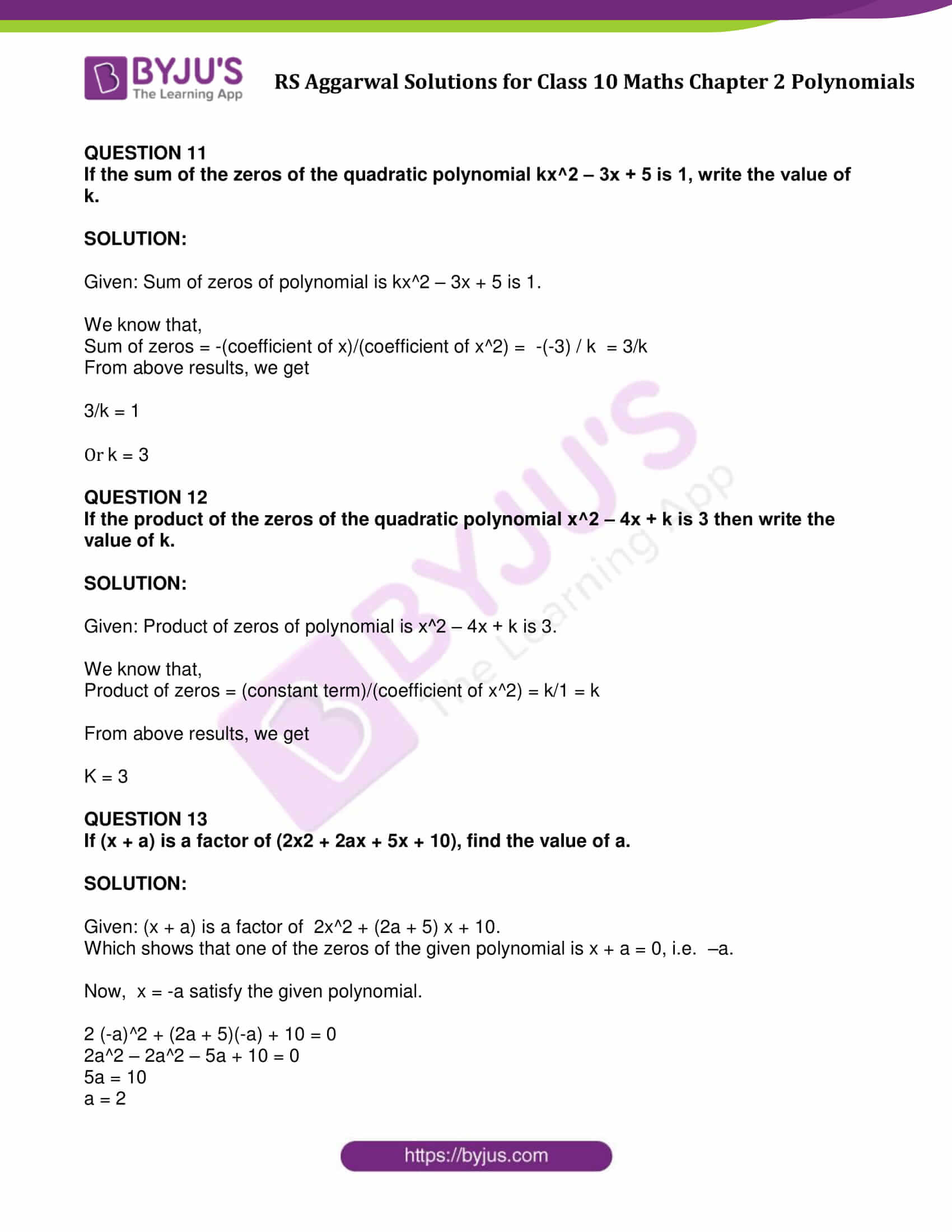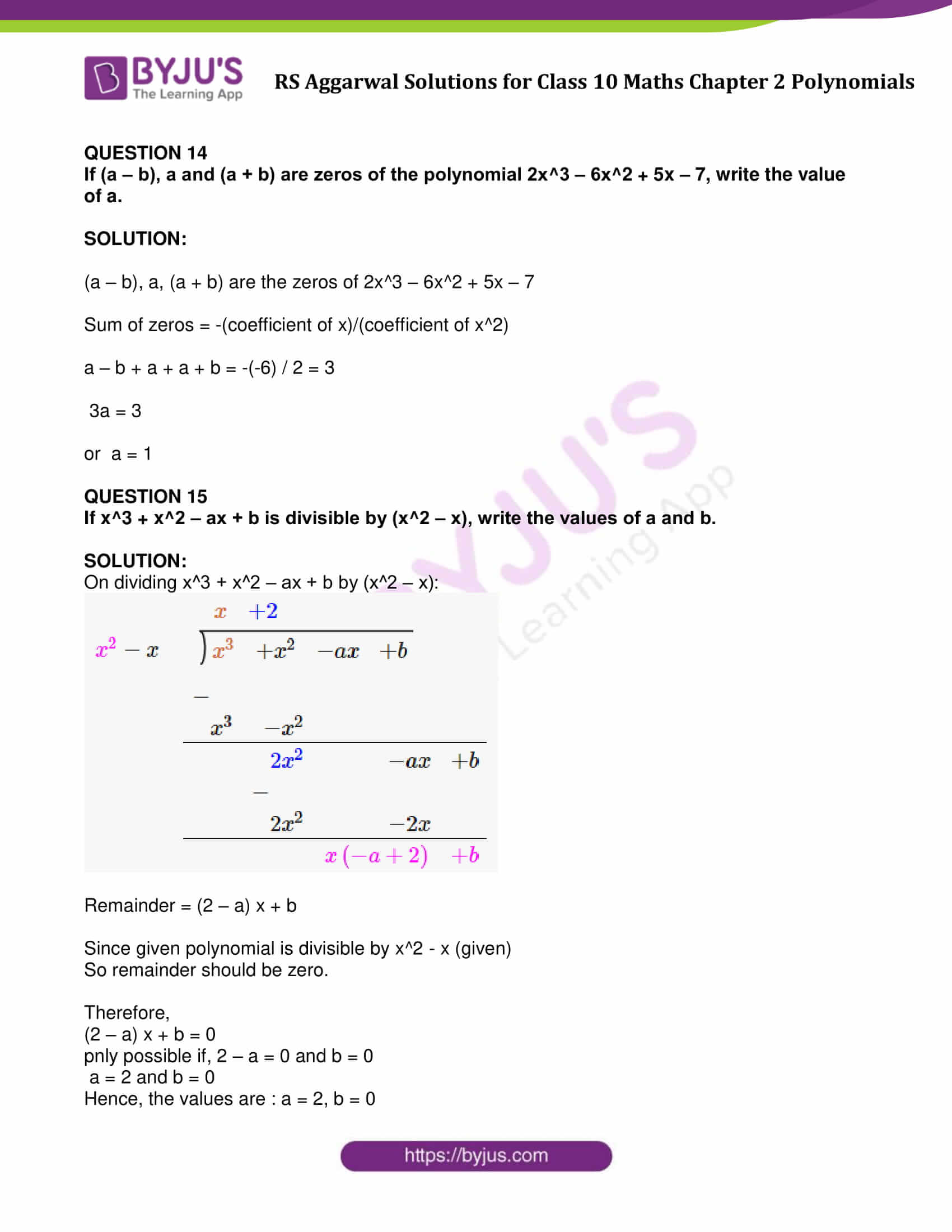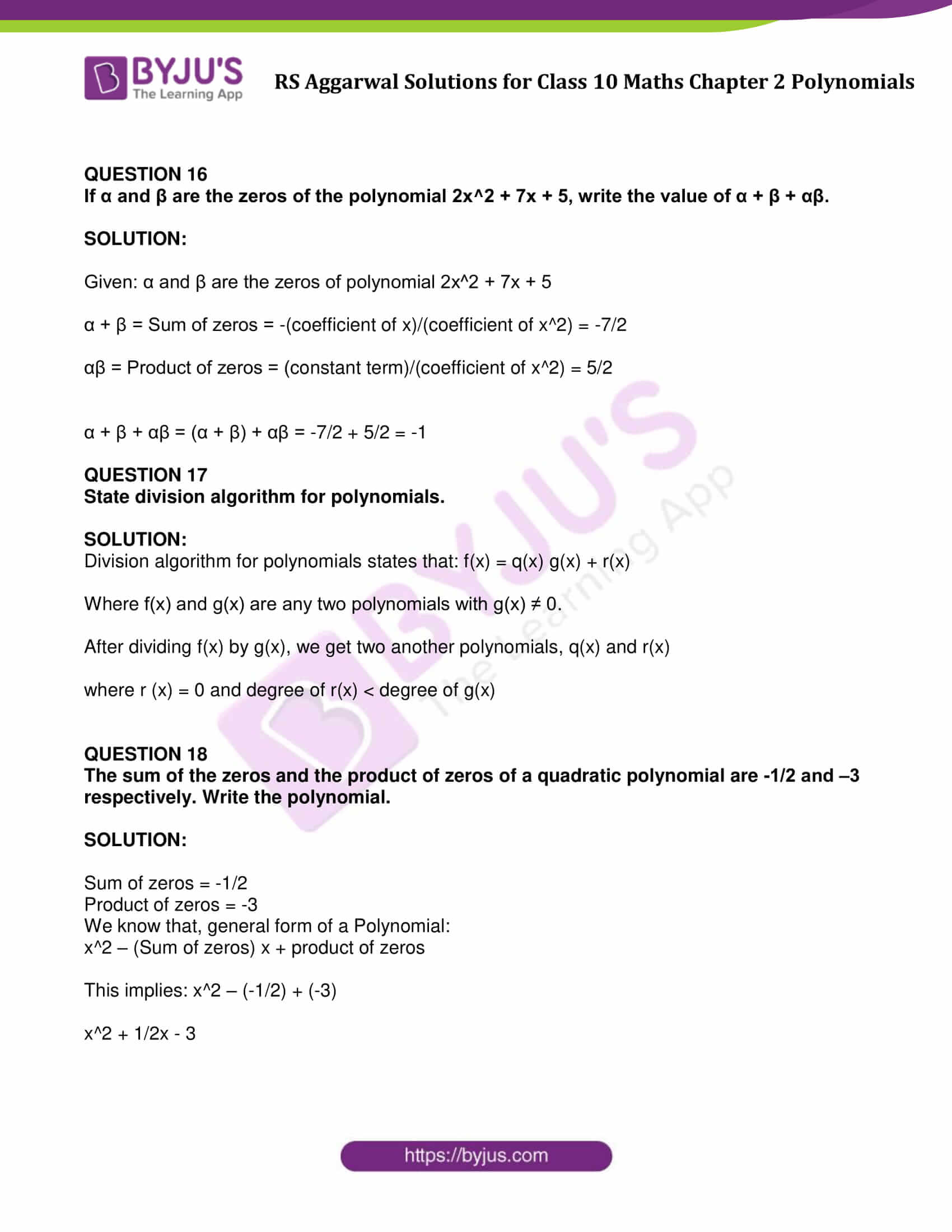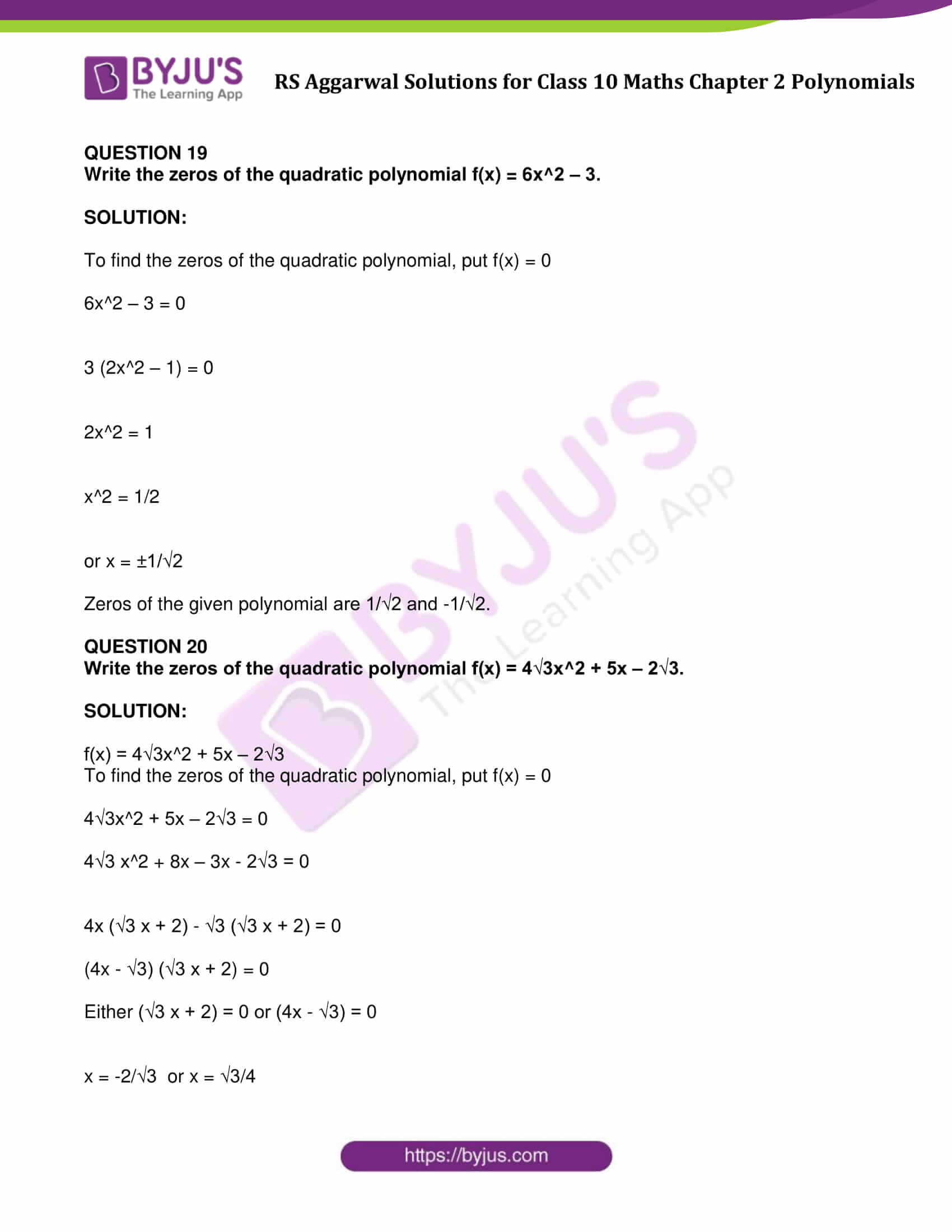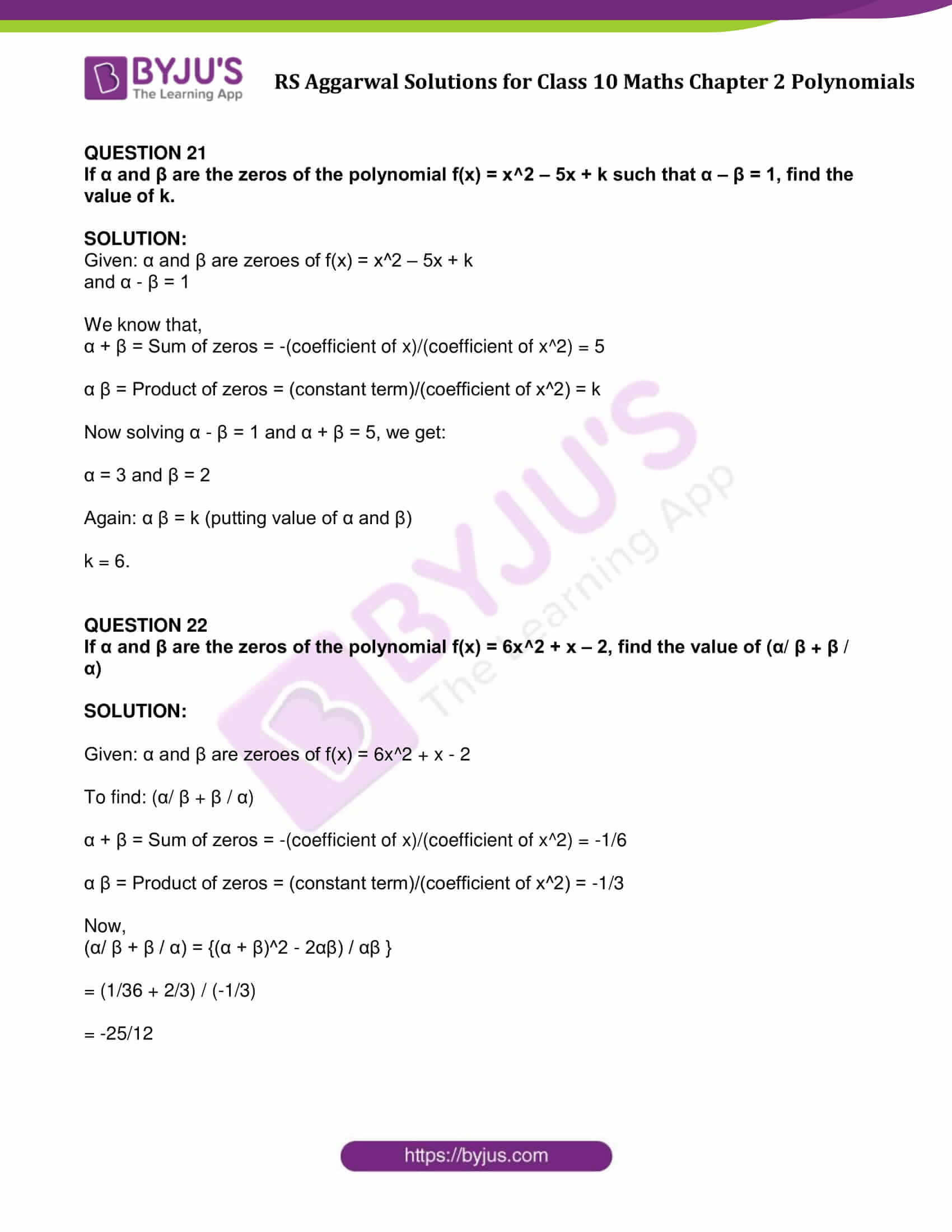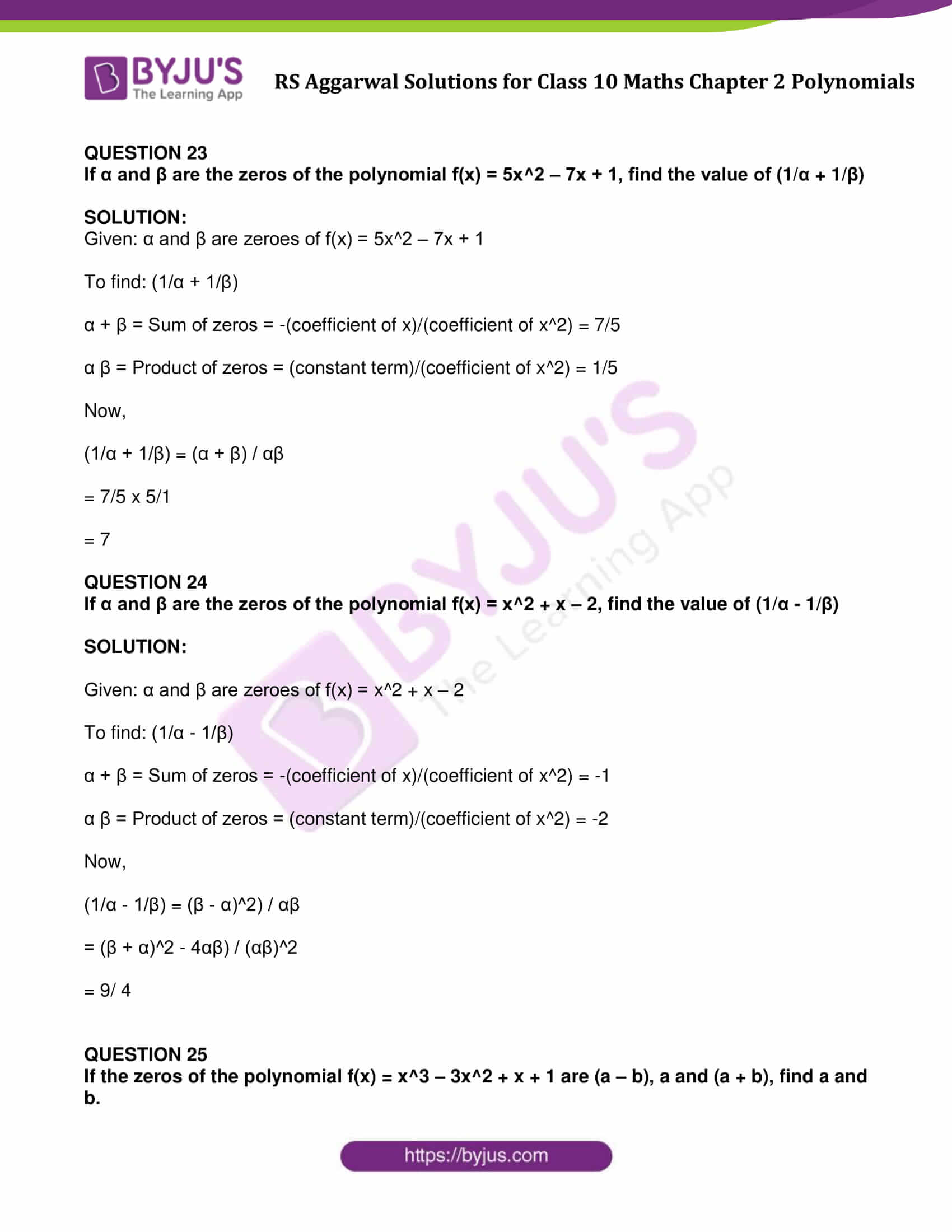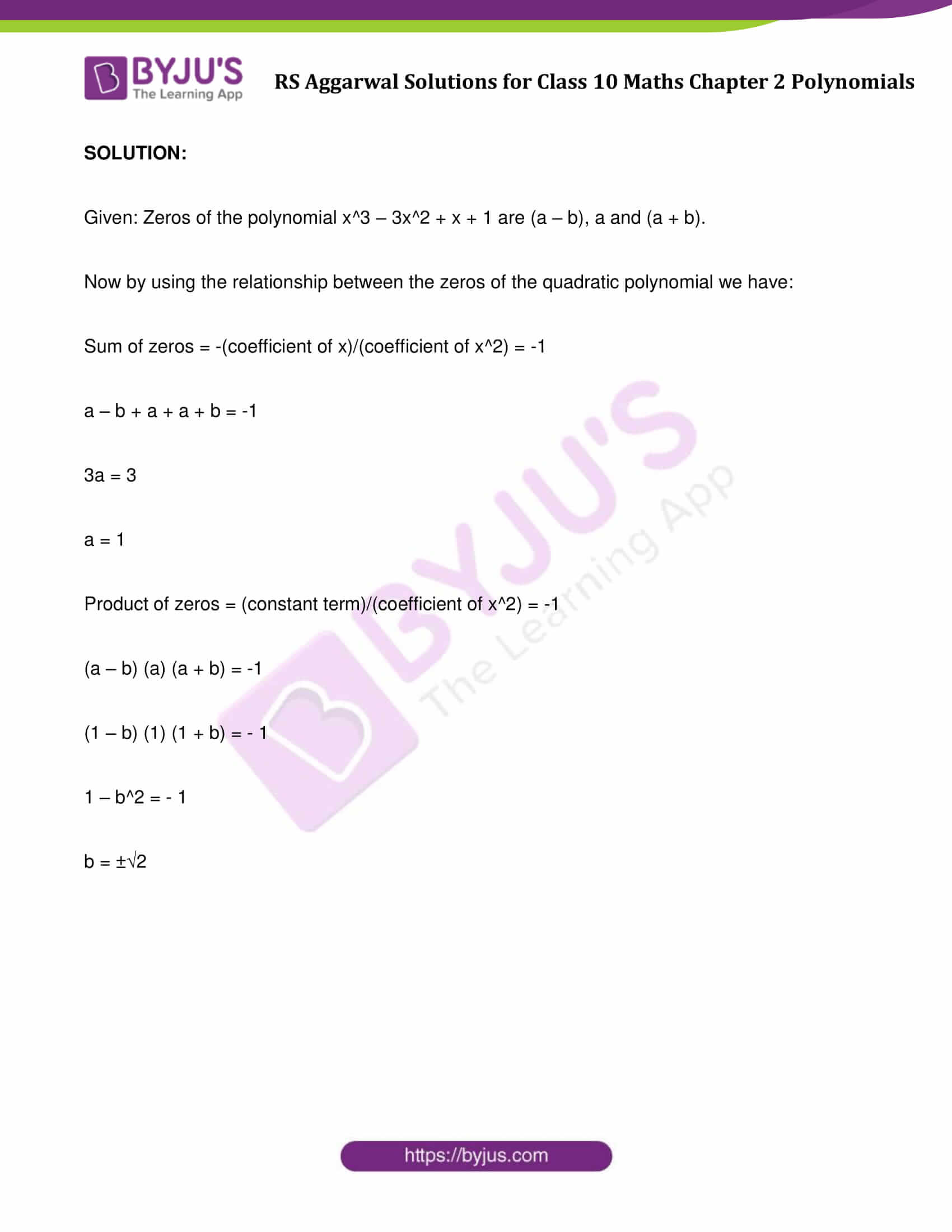## RS Aggarwal Solutions For Class 10 Maths Chapter 2 Exercises:

Get detailed solutions for all the questions listed under the below exercises:

Exercise 2A Solutions: 21 Questions (Short Answers)

Exercise 2B Solutions: 19 Questions (Short Answers)

Exercise 2C Solutions: 25 Questions (Short Answers)

## Exercise 2A Page No: 48

Find the zeros of the following quadratic polynomials and verify the relationship between the zeros and the coefficients:

x^2 + 7x + 12

• x^2 ˗ 2x ˗ 8
• x^2 + 3x ˗ 10
• 4x^2 ˗ 4x ˗ 3
• 5x^2 ˗ 4 ˗ 8x
• 2 √3 x^2 – 5x + √3
• 2x^2 ˗ 11x + 15
• 4x^2 – 4x + 1
• (x^2 ˗ 5)
• (8x^2 ˗ 4)
• (5y^2 + 10y)

(3x^2 – x – 4)Solution:1. x^2 + 7x + 12

Let f(x) = x^2 + 7x + 12

f(x) = x^2 + 4x + 3x + 12

f(x) = x(x+4) + 3(x+4)

f(x) = (x+4)(x+3)

To find the zeroes, set f(x) = 0, then

either (x + 4) = 0 or (x + 3) = 0

x = −4 or x = −3

Again,

Sum of zeroes = (-4 – 3) = -7 = -7/1

= -b/a

= (-Coefficient of x)/(Cofficient of x^2)

Product of zeroes = 12 = 12/1

= c/a

= Constant term / Coefficient of x^2

2. x^2 – 2x – 8

Let f(x) = x^2 ˗ 2x ˗ 8

= x^2 ˗ 4x + 2x ˗ 8

= x(x ˗ 4) + 2(x ˗ 4)

= (x ˗ 4) (x + 2)

To find the zeroes, set f(x) = 0, then

either (x ˗ 4) = 0 or (x+2) = 0

x = 4 or x = -2

Again,

Sum of zeroes = (4 – 2) = 2 = 2/1

= -b/a

= (-Coefficient of x)/(Cofficient of x^2)

Product of zeroes = (4) (-2) = -8/1

= c/a

= Constant term / Coefficient of x^2

3. x^2 + 3x ˗ 10

Let f(x) = x^2 + 3x ˗ 10

= x^2 + 5x ˗ 2x ˗ 10

= x(x + 5) ˗ 2(x + 5)

= (x ˗ 2) (x + 5)

To find the zeroes, set f(x) = 0, then

either x ˗ 2 = 0 or x + 5 = 0

⇒ x = 2 or x = −5.

So, the zeroes of f(x) are 2 and −5.

Again,

Sum of zeroes = 2 + (-5) = -3 = (-3)/1

= -b/a

= (-Coefficient of x)/(Cofficient of x^2)

Product of zeroes = (2)(-5) = -10 = (-10)/1

= c/a

= Constant term / Coefficient of x^2

4. 4x^2 ˗ 4x – 3

Let f(x) = 4x^2 ˗ 4x ˗ 3

= 4x^2 ˗ (6x ˗ 2x) ˗ 3

= 4x^2 ˗ 6x + 2x ˗ 3

= 2x (2x ˗ 3) + 1(2x ˗ 3)

= (2x + 1) (2x ˗ 3)

To find the zeroes, set f(x) = 0

(2x + 1) (2x ˗ 3)= 0

2x + 1 = 0 or 2x ˗ 3 = 0

x = -1/2 or x = 3/2

Again,

Sum of zeroes = (-1/2) + (3/2) = (-1+3)/2 = 2/2

= -b/a

= (-Coefficient of x)/(Cofficient of x^2)

Product of zeroes = (-1/2)(3/2) = (-3)/4

= c/a

= Constant term / Coefficient of x^2

5. 5x^2 ˗ 4 ˗ 8x

Let f(x) = 5x^2 ˗ 4 ˗ 8x

= 5x^2 ˗ 8x ˗ 4

= 5x^2 ˗ (10x ˗ 2x) ˗ 4

= 5x^2 ˗ 10x + 2x ˗ 4

= 5x (x ˗ 2) + 2(x ˗ 2)

= (5x + 2) (x ˗ 2)

To find the zeroes, set f(x) = 0

(5x + 2) (x ˗ 2) = 0

5x + 2 = 0 or x ˗ 2 = 0

x = (−2)/5 or x = 2

Again,

Sum of zeroes = (-2)/5 + 2 = (-2+10)/5 = 8/5

= -b/a

= (-Coefficient of x)/(Cofficient of x^2)

Product of zeroes = (-2/5) x 2 = (-4)/5

= c/a

= Constant term / Coefficient of x^2

6. 2 √3 x^2 – 5x + √3

Let f(x) = 2 √3 x^2 – 5x + √3

= 2 √3 x^2 – 2x – 3x + √3

= 2x(√3x-1) – √3(√3x-1)

To find the zeroes, set f(x) = 0

(√3x – 1) or (2x – √3) = 0

x = 1/√3 = √3/3 or x = √3/2

x = √3/3 or x = √3/2

Again,

Sum of zeroes = √3/3 + √3/2 = 5√3/6

= -b/a

= (-Coefficient of x)/(Cofficient of x^2)

Product of zeroes = √3/3 x √3/2 = √3/6

= c/a

= Constant term / Coefficient of x^2

7. 2x^2 – 11x + 15

Let f(x) = 2x^2 ˗ 11x + 15

= 2x^2 ˗ (6x + 5x) + 15

= 2x_2 ˗ 6x ˗ 5x + 15

= 2x (x ˗ 3) ˗ 5 (x ˗ 3)

= (2x ˗ 5) (x ˗ 3)

To find the zeroes, set f(x) = 0

(2x ˗ 5) (x ˗ 3) = 0

2x ˗ 5= 0 or x ˗ 3 = 0

x = 5/2 or x = 3

Again,

Sum of zeroes = 5/2 + 3 = (5+6)/2 = 11/2

= -b/a

= (-Coefficient of x)/(Cofficient of x^2)

Product of zeroes = 5/2 x 3 = 15/2

= c/a

= Constant term / Coefficient of x^2

8. 4x^2 ˗ 4x + 1

Let f(x) = 4x^2 ˗ 4x + 1

= (2x^2) – 2(2x)(1) + (1)^2

= (2x – 1)^2

To find the zeroes, set f(x) = 0

(2x – 1)^2 = 0

x = 1/2 or x = 1/2

Again,

Sum of zeroes = 1/2+1/2=1=1/1

= -b/a

= (-Coefficient of x) / (Cofficient of x^2)

Product of zeroes = 1/2 x 1/2=1/4

= c/a

= Constant term / Coefficient of x^2

9. (x^2 – 5)

Let f(x) = (x^2 – 5)

= (x^2-(√5)^2)

= (x + √5)(x – √5)

To find the zeroes, set f(x) = 0

(x + √5)(x – √5)=0

x = -√5 or x = √5

Again,

Sum of zeroes = -√5 + √5 = 0/1

= -b/a

= (-Coefficient of x)/(Cofficient of x^2)

Product of zeroes = -√5 + √5 = -5 = -5/1

= c/a

= Constant term / Coefficient of x^2

10. (8x^2 ˗ 4)

Let f(x) = 8x^2 – 4

= 4 ((√2x)^2 – (1)^2)

= 4(√2x + 1)(√2x – 1)

To find the zeroes, set f(x) = 0

(√2x + 1)(√2x – 1) = 0

(√2x + 1) = 0 or (√2x – 1) = 0

x = (-1)/√2 or x = 1/√2

So, the zeroes of f(x) are (-1)/√2 and x = 1/√2

Again,

Sum of zeroes = -1/√2 + 1/√2 = (-1+1)/√2 = 0

= -b/a

= (-Coefficient of x)/(Cofficient of x^2)

Product of zeroes = -1/√2 x 1/√2 = -1/2 = -4/8

= c/a

= Constant term / Coefficient of x^2

11. (5y^2 + 10y)

Let f(x) = (5y^2 + 10y)

= 5y (y + 2)

To find the zeroes, set f(x) = 0

5y (y + 2) = 0

y = 0 or y = -2

So, the zeroes of f(x) are 0 and -2

Again,

Sum of zeroes = -2 + 0 = -2 = -10/5

= -b/a

= (-Coefficient of x)/(Cofficient of x^2)

Product of zeroes = -2 x 0 = 0

= c/a

= Constant term / Coefficient of x^2

12. (3x^2 ˗ x ˗ 4)

Let f(x) = 3x^2 ˗ x ˗ 4

3x^2 ˗ 4x + 3x ˗ 4

= x(3x ˗ 4) + 1 (3x ˗ 4)

= (3x ˗ 4) (x + 1)

To find the zeroes, set f(x) = 0

(3x ˗ 4) = 0 or (x + 1) = 0

⇨ x = 4/3 or x=-1

So, the zeroes of f(x) are 4/3 and x=-1

Again,

Sum of zeroes = 4/3 + (-1) = 1/3

= -b/a

= (-Coefficient of x)/(Cofficient of x^2)

Product of zeroes = 4/3 + (-1) = -4/3

= c/a

= Constant term / Coefficient of x^2

13. Find the quadratic polynomial whose zeroes are 2 and -6. Verify the relation between the coefficients and the zeroes of the polynomial.

Solution:

Let ∝ = 2 and β = -6

Sum of the zeroes = (∝ + β) = 2 – 6 = -4

Product of the zeroes, 𝛼𝛽 = 2(-6) = -12

⇨ x^2 – (∝+β)x + ∝β = x^2 – (-4)x – 12

= x^2 + 4x – 12

And,

Sum of the zeroes = – 4 = -4/1 = (-Coefficient of x)/(Cofficient of x^2)

Product of zeroes = -12 = -12/1 = Constant term / Coefficient of x^2

14. Find the quadratic polynomial whose zeroes are 2/3 and -1/4. Verify the relation between the coefficients and the zeroes of the polynomial.

Solution:

Let ∝ = 2/3 and β = -1/4

Sum of the zeroes = (∝ + β) = 2/3 + -1/4 = 5/12

Product of the zeroes, 𝛼𝛽 = 2/3 x -1/4 = -1/6

⇨ x^2 – (∝+β)x + ∝β = x^2 – (5/12)x – (-1/6)

= 1/12(12x^2 – 5x – 2)

And,

Sum of the zeroes = 5/12 = (-Coefficient of x)/(Cofficient of x^2)

Product of zeroes = -1/6 = Constant term / Coefficient of x^2

15. Find the quadratic polynomial, sum of whose zeroes is 8 and their product is 12. Hence, find the zeroes of the polynomial.

Solution:

Given : Sum of zeroes = (∝ + β) = 8

Product of the zeroes = 𝛼𝛽 = 12

x^2 – (∝+β)x + ∝β = x^2 – (8)x + 12

Now, find the zeroes of the above polynomial.

Let f(x) = x^2 – (8)x + 12

= x^2 – 6x – 2x + 12

= (x -6)(x – 2)

Substitute f(x) = 0.

either (x -6) = 0 or (x – 2) = 0

⇨ x = 6 or x = 2

2 and 6 are the zeroes of the polynomial.

16. Find the quadratic polynomial, sum of whose zeroes is 0 and their product is -1. Hence, find the zeroes of the polynomial.

Solution:

Given : Sum of zeroes = (∝ + β) = 0

Product of the zeroes = 𝛼𝛽 = -1

x^2 – (∝+β)x + ∝β = x^2 – (0)x – 1

= x^2 – 1

Now, find the zeroes of the above polynomial.

Let f(x) = x^2 – 1

= x^2 – 1^2

= (x – 1)(x + 1)

Substitute f(x) = 0.

either (x – 1) = 0 or (x + 1) = o

⇨ x = 1 or x = -1

1 and -1 are the zeroes of the polynomial.

17. Find the quadratic polynomial, sum of whose zeroes is 5/2 and their product is 1. Hence, find the zeroes of the polynomial.

Solution:

Given : Sum of zeroes = (∝ + β) = 5/2

Product of the zeroes = 𝛼𝛽 = 1

x^2 – (∝+β)x + ∝β = x^2 – (5/2)x + 1

= 1/2(2x^2 – 5x + 2)

Now, find the zeroes of the above polynomial.

Let f(x) = 1/2(2x^2 – 5x + 2)

= 1/2(2x^2 – 4x – x + 2)

= 1/2(2x(x – 2) – (x – 2))

= 1/2( (2x – 1)(x – 2) )

Substitute f(x) = 0.

1/2( (2x – 1)(x – 2) ) = 0

either (2x – 1) = 0 or (x – 2) = o

⇨ x = 1/2 or x = 2

1/2 and 2 are the zeroes of the polynomial.

18. Find the quadratic polynomial, sum of whose roots is √2 and their product is 1/3.

Solution:

Given : Sum of zeroes = (∝ + β) = √2

Product of the zeroes = 𝛼𝛽 = 1/3

x^2 – (∝+β)x + ∝β = x^2 – (√2)x + 1/3

= x^2 – √2x + 1/3

19. If x = 2/3 and x = -3 are the roots of the quadratic equation ax^2 + 7x + b = 0 then find the values of a and b.

Solution:

Given roots are: x = 2/3 and x = -3

and quadratic equation ax^2 + 7x + b = 0

Since x = 2/3 and x = -3 are roots of the above quadratic equation

Hence, will satisfy the given equation.

Step 1: At x = 2/3

a(2/3)^2 + 7(2/3) + b=0

4/9 a + 14/3 + b = 0

4a + 42 + 9b = 0 ………….equation (1)

Step 2: At x = –3

a(-3)^2+7(-3)+b=0

9a – 21 + b = 0 ………….equation (2)

Step 3: Solving equation (1) and equation (2), we get

a = 3, b = –6

20. If (x + a) is a factor of the polynomial 2x^2 + 2ax + 5x + 10, find the value of a.

Solution:

Given: (x + a) is a factor of polynomial 2x^2 + 2ax + 5x + 10.

So, we have

x + a = 0

or x = –a , will satisfy the given polynomial.

Therefore, we will have

2 (–a)^2 + 2a(–a) + 5(–a) + 10 = 0

2a^2 –2a^2 – 5a + 10 = 0

– 5a = – 10

a = 2

The value of a is 2.

21. One zero of the polynomial 3x^3 + 16x^2 + 15x – 18 is 2/3. Find the other zeros of the polynomial.

Solution:

Given: x = 2/3 is one of the zero of polynomial 3x^3 + 16x^2 + 15x – 18

Now, we have

x = 2/3

or x – 2/3 = 0

To find other zeroes, let us divide the polynomial 3x^3 + 16x^2 + 15x – 18 by x – 2/3.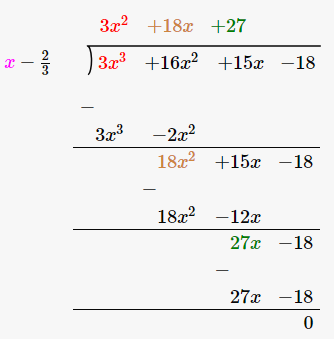So, the quotient is 3x^2 + 18x + 27

Again, set 3x^2 + 18x + 27 = 0 to find the other zeroes.

3x^2 + 18x + 27 = 0

3x^2 + 9x + 9x + 27 = 0

3x(x + 3) + 9(x + 3) = 0

(x + 3) (3x + 9) = 0

Either (x + 3) = 0 or (3x + 9) = 0

x = -3 or x = -3

## Exercise 2B Page No: 58

Question 1: Verify that 3, -2, 1 are the zeros of the cubic polynomial p(x) = (x^3 – 2x^2 – 5x + 6) and verify the relation between it zeros and coefficients.

Solution:

Let f(x) = x^3 – 2x^2 – 5x + 6

3, -2 and 1 are the zeros of the polynomial (given)

Therefore,

f(3) = (3)^3 – 2(3)^2 – 5(3) + 6

= 27 – 18 – 15 + 6

= 0

f(-2) = (-2)^3 – 2(-2)^2 – 5(-2) + 6

= -8 – 8 + 10 + 6

= 0

f(1) = (1)^3 – 2 (1)^2 – 5 (1) + 6

= 1 – 2 – 5 + 6

= 0

Verify relations:

General form of a cubic equation: ax^3 + bx^2 + cx + d.

Now,

Consider α = 3, β = – 2 and γ = 1

α + β + γ = 3 – 2 + 1 = 2 = -b/a

αβ + βγ + αγ = 3 (-2) + (-2) (1) + 1 (3) = -5 = c/a

And, αβγ = 3 (-2)(1) = -6 = -d/a

Question 2: Verify that 5, -2 and 1/3 are the zeroes of the cubic polynomial p(x) = (3x^3 – 10x^2 – 27x + 10) and verify the relation between its zeroes and coefficients.

Solution:

Let f(x) = 3x^3 – 10x^2 – 27x + 10

5, -2 and 1/3 are the zeros of the polynomial (given)

Therefore,

f(5) = 3(5)^3 – 10(5)^2 – 27(5) + 10

= 3 × 125 – 250 – 135 + 10

= 0

f(-2) = 3(-2)^3 – 10(-2)^2 – 27(-2) + 10

= – 24 – 40 + 54 + 10

= 0

f(1/3) = 3 (1/3)^3 – 10(1/3)^2 – 27(1/3) + 10

= 1/8 – 10/9 – 9 + 10

= 0

Verify relations:

General form of a cubic equation: ax^3 + bx^2 + cx + d.

Now,

Consider α = 5, β = – 2 and γ = 1/3

α + β + γ = 5 – 2 + 1/3 = 10/3 = -b/a

αβ + βγ + αγ = 5 (-2) + (-2) (1/3) + (1/3) (5) = -9 = c/a

And, αβγ = 5 (-2) (1/3)= -10/3 = -d/a

Question 3: Find a cubic polynomial whose zeroes are 2, -3 and 4.

Solution:

General form of a cubic polynomial whose zeroes are a, b and c is:

x^3 – (a + b + c) x^2 + (ab + bc + ca)x – abc …(1)

To Find: Cubic polynomial whose zeroes are 2, -3 and 4

Let us say, a = 2, b = – 3 and c = 4

Putting the values of a, b and c in the equation (1) we get:

= x^3 – (2 – 3 + 4) x^2 + (-6 – 12 + 8) x – (- 24)

= x^3 – 3x^2 – 10x + 24

Which is required polynomial.

Question 4: Find a cubic polynomial whose zeroes are 1/2, 1 and -3.

Solution:

General form of a cubic polynomial whose zeroes are a, b and c is:

x^3 – (a + b + c) x^2 + (ab + bc + ca)x – abc …(1)

To Find: Cubic polynomial whose zeroes are 1/2, 1 and -3

Let us say, a = 1/2, b = 1 and c = -3

Putting the values of a, b and c in the equation (1) we get:

= x^3 – (1/2 + 1 – 3) x^2 + (1/2 – 3 – 3/2)x – (- 3/2)

= x^3 – (-3/2) x^2 – 4x + 3/2

= 2x^3 + 3x^2 – 8x + 3

Which is required polynomial.

Question 5: Find a cubic polynomial with the sum of its zeroes, sum of the products of its zeroes taken two at a time and the product of its zeroes as 5, -2 and -24 respectively.

Solution:

As we know, General form of a cubic polynomial whose zeroes are a, b and c is:

x^3 – (a + b + c) x^2 + (ab + bc + ca)x – abc

Also written as :

x^3 – (Sum of the zeros) x^2 + (Sum of the product of the zeros taking two at a time) x – (Product of Zeros) …(1)

Given:

Sum of the zeros = 5

Sum of the product of its zeros taken two at a time -2

Product of its zeros = –24

Putting these values in the equation (1), we get:

x^3 – 5x^2 – 2x + 24

Which is required polynomial.

Question 6: Find the quotient and remainder when

f(x) = x^3-3x^2+5x-3 is divided by g(x)= x^2-2

Solution:

Divide f(x) = x^3-3x^2+5x-3 by g(x)= x^2-2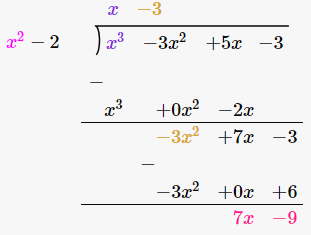Quotient = x – 3

Remainder = 7x – 9

Question 7: Find the quotient and remainder when

f(x) = x^4 – 3x^2 + 4x + 5 is divided by g(x)= x^2 + 1 – x

Solution:

f(x) = x^4 – 3x^2 + 4x + 5 divide by g(x)= x^2 + 1 – x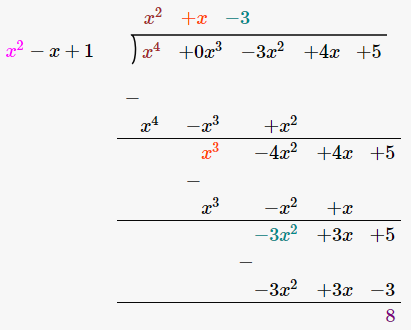Quotient = x^2 + x – 3

Remainder = 8

Question 8: Find the quotient and remainder when

f(x) = x^4 – 5x + 6 is divided by g(x) = 2 – x^2

Solution: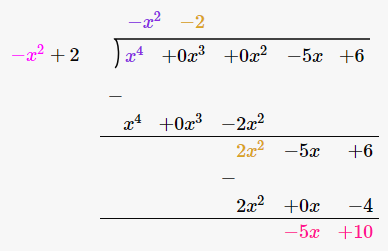Quotient = -x^2 – 2

Remainder = -5x + 10

Question 9: By actual division, show that x2 – 3 is a factor of 2x^4 + 3x^3 – 2x^2 – 9x – 12.

Solution:

x2 – 3 is a factor of 2x^4 + 3x^3 – 2x^2 – 9x – 12 only if remainder is zero.

Divide 2x^4 + 3x^3 – 2x^2 – 9x – 12 by x2 – 3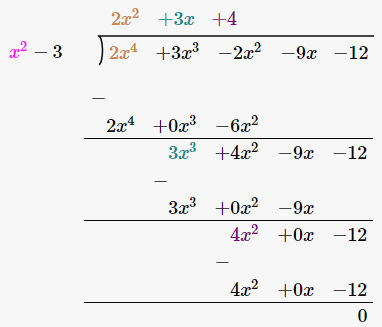Question 10: On dividing 3x^3 + x^2 + 2x + 5 is divided by a polynomial g(x), the quotient and remainder are (3x – 5) and (9x + 10) respectively. Find g(x).

Solution:

As per Division Rule:

Dividend = Quotient × Divisor + Remainder …(i)

Given :

Dividend = 3x^3 + x^2 + 2x + 5

Quotient = 3x – 5

Remainder = 9x + 10

(i) implies:

(3x^3 + x^2 + 2x + 5) / (3x – 5) = g (x)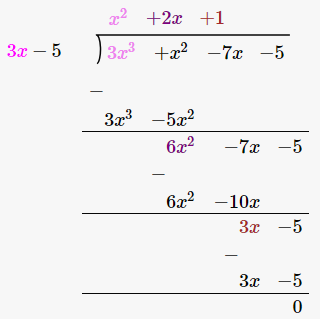Therefore g(x) = x^2 + 2x + 1.

Question 11: Verify division algorithm for the polynomial f(x) = 8 + 20x + x^2 – 6x^3 by g(x) = 2 + 5x – 3x^2.

Solution:

f(x) = -6x^3 + x^2 + 20x + 8

g(x) = -3x^2 + 5x + 2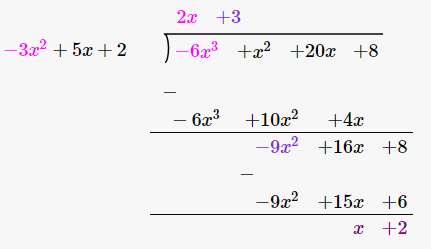Quotient = 2x + 3

Remainder = x + 2

Verification:

As per division rule:

Dividend = Quotient x Divisor + Remainder

After Putting the values, we get:

– 6x^3 + x^2 + 20x + 8 = (- 3x^2 + 5x + 2) (2x + 3) + (x + 2)

-6x^3 + x^2 + 20x + 8 = -6x^3 + x^2 + 20x + 8

Question 12: It is given that -1 is one of the zeroes of the polynomial x^3 + 2x^2 – 11x – 12 . Find all the zeroes of the given polynomial.

Solution:

Let f (x) = x^3 + 2x^2 ‒ 11x ‒ 12

Given: -1 is a zero of the polynomial, which means (x + 1) is a factor of f (x).

Dividing f (x) by (x + 1), we get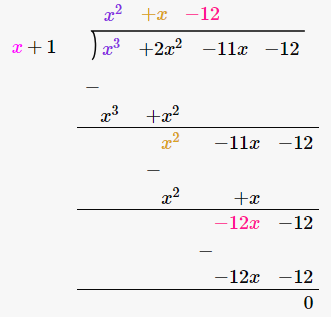f (x) becomes:

f(x) = (x + 1) (x^2 + x – 12)

= (x + 1) (x^2 + 4x – 3x – 12)

= (x + 1) (x – 3) (x + 4)

If f (x) = 0

• (x + 1) (x – 3) (x + 4) = 0

Either (x + 1) = 0 or (x – 3) = 0 or (x + 4) = 0

x = -1 or x = 3 or x = – 4

Zeros of the polynomial are -1, 3 and -4

Question 13: If 1 and –2 are two zeroes of the polynomial (x^3 – 4x^2 – 7x + 10) , find its third zero.

Solution:

Let f(x) = x^3 – 4x^2 – 7x + 10

Given: 1 and – 2 are the zeros of the given polynomial therefore (x – 1) and (x + 2) is are factors of f (x).

Consequently, (x – 1) (x + 2) = (x^2 + x – 2) is a factor of f(x).

Divide x^3 – 4x^2 – 7x + 10 by (x^2 + x – 2):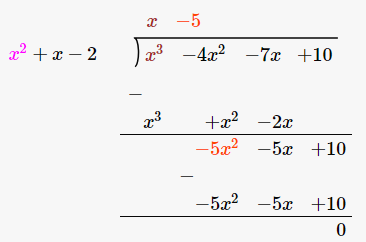Put f (x) = 0

(x^2 + x – 2) (x – 5) = 0

(x – 1) (x + 2) (x – 5) = 0

x = 1 or x = – 2 or x = 5

Hence, the third zero is 5.

Question 14: If 3 and –3 are two zeroes of the polynomial (x^4 + x^3 – 11x^2 – 9x + 18), find all the zeroes of the given polynomial.

Solution:

Let f(x) = x^4 + x^3 – 11x^2 – 9x + 18

Given: 3 and – 3 are the zeros of the polynomial, (x + 3) and (x – 3) are factors of f (x), and

Consequently, (x – 3) (x + 3) = (x^2 – 9) is a factor of f (x)

Divide f(x) by (x^2 – 9) we get: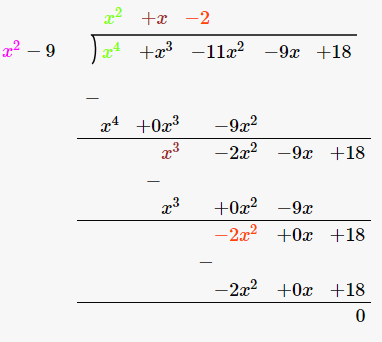Put f(x) = 0

(x^2 + x – 2) (x^2 – 9) = 0

(x – 1) (x + 2) (x – 3) (x + 3) = 0

x = 1 or x = – 2 or x = 3 or x = – 3

Hence, all the zeros of the given polynomial are 1, -2, 3 and -3

Question 15: If 2 and -2 are two zeroes of the polynomial (x^4 + x^3 – 34x^2 – 4x + 120), find all the zeroes of the given polynomial.

Solution:

Let us assume f (x) = x^4 + x^3 – 34x^2 – 4x + 120

Given: 2 and – 2 are the zeros of the polynomial

(x – 2) and (x + 2) are factors of f(x) and

(x – 2) (x + 2) = (x^2 – 4) is a factor of f (x), and

Divide f(x) by (x^2 – 4) we get: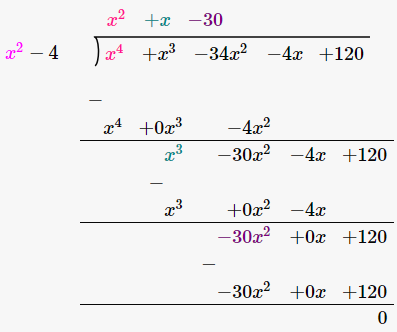Set f (x) = 0

(x^2 + x – 30) (x^2 – 4) = 0

(x^2 + 6x – 5x – 30) (x – 2)(x + 2) = 0

[x (x + 6) – 5 (x + 6)] (x – 2) (x + 2) = 0

(x – 5) (x + 6) (x – 2) (x + 2) = 0

either x = 5 or x = – 6 or x = 2 or x = – 2

Hence, all the zeros of the given polynomial are 2, -2, 5 and -6.

Question 16: Find all the zeroes of x^4 + x^3 – 23x^2 – 3x + 60, if it is given that two of its zeroes are √3 and – √3 .

Solution:

Let f(x) = x^4 + x^3 – 23x^2 – 3x + 60

Given: √3 and –√3 are the zeros of the polynomial.

(x – √3) and (x – √3) are factors of f(x)

So, (x – √3) (x + √3) = (x^2 – 3) is a factor of f(x)

Divide f(x) by (x^2 – 3):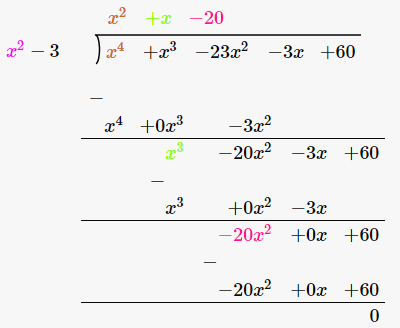Set f(x) = 0

(x^2 + x – 20) (x^2 – 3) = 0

(x^2 + 5x – 4x – 20) (x^2 – 3) =0

[x (x + 5) – 4 (x + 5)] (x2 – 3)=0

(x – 4) (x + 5) (x – ) (x + ) = 0

either x = 4 or x = – 5 or x = √3 or x = – √3

Hence, all the zeros of the given polynomial are √3, -√3, 4 and – 5

Question 17: Find all the zeroes of (2x^4 – 3x^3 – 5x^2 + 9x – 3) , it is being given that two of its zeroes are √3 and – √3.

Solution:

Let f(x) = 2x^4 – 3x^3 – 5x^2 + 9x – 3

Given: √3 and –√3 are the zeros of the polynomial.

(x – √3) and (x – √3) are factors of f(x)

So, (x – √3) (x + √3) = (x^2 – 3) is a factor of f(x)

Divide f(x) by (x^2 – 3):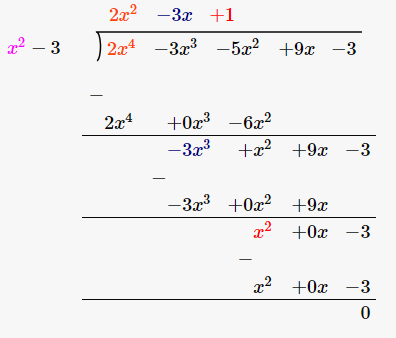Set f(x) = 0

(2x^2 – 3x + 1) (x^2 – 3) = 0

2x^2 – 3x^2 – 5x^2 + 9x – 3 = 0

(x^2 – 3) (2x^2 – 3x + 1) = 0

(x^2 – 3) (2x^2 – 2x – x + 1) (2x – 1) (x – 1) = 0

(x – √3) (x + √3) (2x – 1) (x – 1) = 0

either x = √3 or x = -√3 or x = 1/2 or x = 1

Hence, all the zeros of the given polynomial are √3 , -√3 , 1/2 and 1

Question 18: Obtain all other zeroes of (x^4 + 4x^3 – 2x^2 – 20x – 15) if two of its zeroes are √5 and –√5.

Solution:

Let f(x) = x^4 + 4x^3 – 2x^2 – 20x – 15

(x-√5) and (x-√5) are the factors of f(x)

and (x-√5) (x + √5) = (x^2 – 5) is a factor of f (x)

Divide f(x) by (x^2 – 5) we get: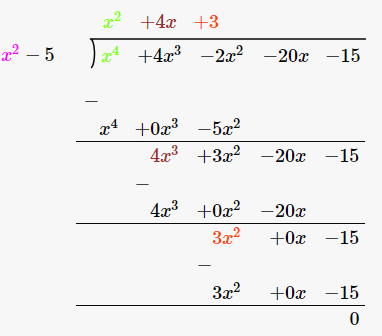Set f(x) = 0

x^4 + 4x^3 – 2x^2 – 20x – 15 = 0

(x^2 – 5) (x^2 + 4x + 3) = 0

(x -√5) (x + √5) (x + 1) (x + 3) = 0

either x = √5 or x = – √5 or x = – 1 or x = – 3

Hence, all the zeros of the given polynomial are √5, -√5, – 1 and – 3.

Question 19: Find all the zeroes of polynomial (2x^4 – 11x^3 + 7x^2 + 13x – 7) , it being given that two of its zeroes are (3 + √2) and (3 – √2).

Solution:

Let f(x) = 2x^4 – 11x^3 + 7x^2 + 13x – 7

Given: (3 + √2) and (3- √2) are the zeros of f(x).

So (x – (3 + √2)) and (x – (3 – √2)) are factors of f(x)

and (x – (3 + √2))(x – (3 – √2)) = x^2 – 6x + 7 is a factor of f(x)

Divide f(x) by x^2 – 6x + 7 we get:Set f(x) = 0

2x^4 – 11x^3 + 7x^2 + 13x – 7 = 0

(x^2 – 6x + 7) (2x^2 + x – 1) = 0

(x + 3 + √2) (x + 3 -√2) (2x – 1) (x + 1) = 0

x = – 3 – √2 or x = – 3 + √2 or x = 1/2 or x = – 1

Hence, all the zeros of the given polynomial are (-3 -√2), (-3 + √2), 1/2 and – 1.

## Exercise 2C Page No: 59

QUESTION 1: If one zero of the polynomial x^2 ‒ 4x + 1 is (2 + √3), write the other zero.

SOLUTION:

Given: (2 + √3) is one of zero of polynomial x^2 ‒ 4x + 1.

To find: Other zero

Since given polynomial is a quadratic so it has only two zeros.

Let other zero be x.

Now,

Sum of zeros = -(coefficient of x)/(coefficient of x^2)

x + (2 + √3) = -(-4/1) = 4

x + 2 + √3 = 4

or x = 2 – √3

Hence, the other zero is (2 -√3).

QUESTION 2: Find the zeros of the polynomial x^2 + x ‒ p (p + 1).

SOLUTION:

Let f(x) = x^2 + x ‒ p (p + 1)

Above polynomial can be written as,

= x^2 + (p + 1) x – px – p (p + 1)

= x (x + (p + 1)) – p (x + (p + 1))

= (x – p)(x + (p + 1))

To find the zeroes of f(x), put f(x) = 0

(x – p)(x + (p + 1)) = 0

either (x – p) = 0 or (x + (p + 1)) = 0

x = p or x = – (p + 1)

Hence, the zeros of the given polynomial are p and – (p + 1)

QUESTION 3

Find the zeros of the polynomial x^2 – 3x – m (m + 3).

SOLUTION:

Let f(x) = x^2 – 3x – m (m + 3)

Above polynomial can be written as,

f(x) = x^2 – (m + 3)x + mx – m(m + 3)

= x(x – m – 3) + m(x – m – 3)

= (x – m – 3)(x + m)

To find the zeroes of f(x), put f(x) = 0

(x – m – 3)(x + m) = 0

Either x – m – 3 = 0 or x + m = 0

x = m + 3 or x = -m

Required Zeros are (m + 3), -m

QUESTION 4

Find α, β are the zeros of a polynomial such that α + β= 6 and αβ = 4 then write the polynomial.

SOLUTION:

Given : α + β= 6 = sum of zeros and

αβ = 4 = Product of zeroes

We know that, if α and β are the zeros of the polynomial then the quadratic polynomial can be x^2 – (α + β) x + αβ

Now substituting the values, we get

x^2 – 6x + 4, which is required polynomial.

QUESTION 5

If one zeros of the quadratic polynomial kx^2 + 3x + k is 2 then find the value of k.

SOLUTION:

Given: 2 is one of zeroes of the polynomial kx^2 + 3x + k , which means x = 2 will satisfy it.

k(2)^2 + 3( 2) + k = 0

4k + 6 + k= 0

5k + 6 = 0

k = -6 / 5

The value of k is -6 / 5

QUESTION 6

If 3 is a zero of the polynomial 2x^2+ x + k, find the value of k.

SOLUTION:

Given: 3 is one of zeroes of the polynomial 2x^2 + x + k , which means x = 3 will satisfy it.

2x^2 + x + k = 0

2(3)^2 + 3 + k = 0

18 + 3+ k = 0

k = -21

the value of k is -21

QUESTION 7

If ‒4 is a zero of the polynomial x^2 ‒ x- (2k + 2) then find the value of k.

SOLUTION:

Given: -4 is one of zeroes of the polynomial x^2 ‒ x- (2k + 2), which means x = -4 will satisfy it.

x^2 – x – (2k + 2) = 0

(-4)^2 – (-4) – 2k – 2 = 0

16 + 4 – 2k – 2 = 0

-2k + 18 = 0

k = 9

The value of k is 9.

QUESTION 8

If 1 is a zero of the polynomial ax^2 ‒ 3 (a ‒ 1) x ‒ 1 then find the value of a.

SOLUTION:

Given: 1 is one of zeroes of the polynomial ax^2 ‒ 3 (a ‒ 1) x ‒ 1 , which means x = 1 will satisfy it.

a(1)^2 – 3(a – 1) x 1 – 1 = 0

a – 3a + 3 – 1 = 0

-2a + 2 = 0

a = 1

The value of a is 1.

QUESTION 9

If ‒2 is a zero of the polynomial 3x^2 + 4x + 2k then find the value of k.

SOLUTION:

Given: -2 is one of zeroes of the polynomial 3x^2 + 4x + 2k , which means x = -2 will satisfy it.

3(-2)^2 + 4(-2) + 2k = 0

12 – 8 + 2k = 0

4 + 2k=0

k = -2

The value of k is -2.

QUESTION 10

Write the zeros of the polynomial x^2 ‒ x ‒ 6.

SOLUTION:

Let f(x) = x^2 – x – 6

= x^2 – 3x + 2x – 6

= x(x – 3) + 2(x – 3)

= (x – 3)(x + 2)\

To find the zeros of f(x), let sat f(x) = 0, we get

(x – 3)(x + 2) = 0

Either x – 3 = 0 or x + 2 = 0

x = 3 or x = -2

Therefore, 3, -2 are zeros.

QUESTION 11

If the sum of the zeros of the quadratic polynomial kx^2 ‒ 3x + 5 is 1, write the value of k.

SOLUTION:

Given: Sum of zeros of polynomial is kx^2 – 3x + 5 is 1.

We know that,

Sum of zeros = -(coefficient of x)/(coefficient of x^2) = -(-3) / k = 3/k

From above results, we get

3/k = 1

Or k = 3

QUESTION 12

If the product of the zeros of the quadratic polynomial x^2 ‒ 4x + k is 3 then write the value of k.

SOLUTION:

Given: Product of zeros of polynomial is x^2 ‒ 4x + k is 3.

We know that,

Product of zeros = (constant term)/(coefficient of x^2) = k/1 = k

From above results, we get

K = 3

QUESTION 13

If (x + a) is a factor of (2×2 + 2ax + 5x + 10), find the value of a.

SOLUTION:

Given: (x + a) is a factor of 2x^2 + (2a + 5) x + 10.

Which shows that one of the zeros of the given polynomial is x + a = 0, i.e. –a.

Now, x = -a satisfy the given polynomial.

2 (-a)^2 + (2a + 5)(-a) + 10 = 0

2a^2 – 2a^2 – 5a + 10 = 0

5a = 10

a = 2

QUESTION 14

If (a ‒ b), a and (a + b) are zeros of the polynomial 2x^3 ‒ 6x^2 + 5x – 7, write the value of a.

SOLUTION:

(a – b), a, (a + b) are the zeros of 2x^3 – 6x^2 + 5x – 7

Sum of zeros = -(coefficient of x)/(coefficient of x^2)

a – b + a + a + b = -(-6) / 2 = 3

3a = 3

or a = 1

QUESTION 15

If x^3 + x^2 ‒ ax + b is divisible by (x^2 ‒ x), write the values of a and b.

SOLUTION:

On dividing x^3 + x^2 ‒ ax + b by (x^2 ‒ x):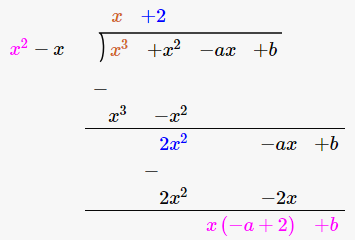Remainder = (2 – a) x + b

Since given polynomial is divisible by x^2 – x (given)

So remainder should be zero.

Therefore,

(2 – a) x + b = 0

pnly possible if, 2 – a = 0 and b = 0

a = 2 and b = 0

Hence, the values are : a = 2, b = 0

QUESTION 16

If α and β are the zeros of the polynomial 2x^2 + 7x + 5, write the value of α + β + αβ.

SOLUTION:

Given: α and β are the zeros of polynomial 2x^2 + 7x + 5

α + β = Sum of zeros = -(coefficient of x)/(coefficient of x^2) = -7/2

αβ = Product of zeros = (constant term)/(coefficient of x^2) = 5/2

α + β + αβ = (α + β) + αβ = -7/2 + 5/2 = -1

QUESTION 17

State division algorithm for polynomials.

SOLUTION:

Division algorithm for polynomials states that: f(x) = q(x) g(x) + r(x)

Where f(x) and g(x) are any two polynomials with g(x) ≠ 0.

After dividing f(x) by g(x), we get two another polynomials, q(x) and r(x)

where r (x) = 0 and degree of r(x) < degree of g(x)

QUESTION 18

The sum of the zeros and the product of zeros of a quadratic polynomial are -1/2 and ‒3 respectively. Write the polynomial.

SOLUTION:

Sum of zeros = -1/2

Product of zeros = -3

We know that, general form of a Polynomial:

x^2 – (Sum of zeros) x + product of zeros

This implies: x^2 – (-1/2) + (-3)

x^2 + 1/2x – 3

QUESTION 19

Write the zeros of the quadratic polynomial f(x) = 6x^2 ‒ 3.

SOLUTION:

To find the zeros of the quadratic polynomial, put f(x) = 0

6x^2 – 3 = 0

3 (2x^2 – 1) = 0

2x^2 = 1

x^2 = 1/2

or x = ±1/√2

Zeros of the given polynomial are 1/√2 and -1/√2.

QUESTION 20

Write the zeros of the quadratic polynomial f(x) = 4√3x^2 + 5x ‒ 2√3.

SOLUTION:

f(x) = 4√3x^2 + 5x ‒ 2√3

To find the zeros of the quadratic polynomial, put f(x) = 0

4√3x^2 + 5x ‒ 2√3 = 0

4√3 x^2 + 8x – 3x – 2√3 = 0

4x (√3 x + 2) – √3 (√3 x + 2) = 0

(4x – √3) (√3 x + 2) = 0

Either (√3 x + 2) = 0 or (4x – √3) = 0

x = -2/√3 or x = √3/4

QUESTION 21

If α and β are the zeros of the polynomial f(x) = x^2 ‒ 5x + k such that α ‒ β = 1, find the value of k.

SOLUTION:

Given: α and β are zeroes of f(x) = x^2 ‒ 5x + k

and α – β = 1

We know that,

α + β = Sum of zeros = -(coefficient of x)/(coefficient of x^2) = 5

α β = Product of zeros = (constant term)/(coefficient of x^2) = k

Now solving α – β = 1 and α + β = 5, we get:

α = 3 and β = 2

Again: α β = k (putting value of α and β)

k = 6.

QUESTION 22

If α and β are the zeros of the polynomial f(x) = 6x^2 + x ‒ 2, find the value of (α/ β + β / α)

SOLUTION:

Given: α and β are zeroes of f(x) = 6x^2 + x – 2

To find: (α/ β + β / α)

α + β = Sum of zeros = -(coefficient of x)/(coefficient of x^2) = -1/6

α β = Product of zeros = (constant term)/(coefficient of x^2) = -1/3

Now,

(α/ β + β / α) = {(α + β)^2 – 2αβ) / αβ }

= (1/36 + 2/3) / (-1/3)

= -25/12

QUESTION 23

If α and β are the zeros of the polynomial f(x) = 5x^2 ‒ 7x + 1, find the value of (1/α + 1/β)

SOLUTION:

Given: α and β are zeroes of f(x) = 5x^2 ‒ 7x + 1

To find: (1/α + 1/β)

α + β = Sum of zeros = -(coefficient of x)/(coefficient of x^2) = 7/5

α β = Product of zeros = (constant term)/(coefficient of x^2) = 1/5

Now,

(1/α + 1/β) = (α + β) / αβ

= 7/5 x 5/1

= 7

QUESTION 24

If α and β are the zeros of the polynomial f(x) = x^2 + x – 2, find the value of (1/α – 1/β)

SOLUTION:

Given: α and β are zeroes of f(x) = x^2 + x – 2

To find: (1/α – 1/β)

α + β = Sum of zeros = -(coefficient of x)/(coefficient of x^2) = -1

α β = Product of zeros = (constant term)/(coefficient of x^2) = -2

Now,

(1/α – 1/β) = (β – α)^2) / αβ

= (β + α)^2 – 4αβ) / (αβ)^2

= 9/ 4

QUESTION 25

If the zeros of the polynomial f(x) = x^3 – 3x^2 + x + 1 are (a – b), a and (a + b), find a and b.

SOLUTION:

Given: Zeros of the polynomial x^3 – 3x^2 + x + 1 are (a – b), a and (a + b).

Now by using the relationship between the zeros of the quadratic polynomial we have:

Sum of zeros = -(coefficient of x)/(coefficient of x^2) = -1

a – b + a + a + b = -1

3a = 3

a = 1

Product of zeros = (constant term)/(coefficient of x^2) = -1

(a – b) (a) (a + b) = -1

(1 – b) (1) (1 + b) = – 1

1 – b^2 = – 1

b = ±√2

## RS Aggarwal Solutions for Class 10 Maths Chapter 2 Polynomials

In this chapter students will study important concepts on polynomials as listed below:

• Linear Polynomials
• Cubic Polynomials
• Value of a Polynomials at a given point
• Zeros of a Polynomials
• Relation between the zeros and coefficients of a quadratic Polynomial

### Key Features of RS Aggarwal Solutions for Class 10 Maths Chapter 2 Polynomials

1. RS Aggarwal Solutions provide fully resolved step by step solutions to all questions.

2. Polynomials study material prepared based on the latest CBSE syllabus by subject experts.

3. Easy for quick revision

4. Help to clear doubts and score good marks in the exams.

5. These solutions will help the students to strengthen their foundation on topic.